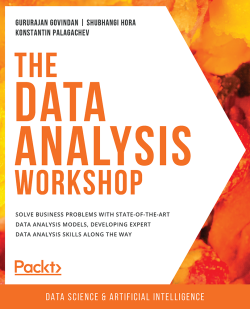# The Data Analysis Workshop3 (2 reviews total)
By Gururajan Govindan , Shubhangi Hora , Konstantin Palagachev
• FREE Subscription Access now
• \$34.99 Print + eBook
• \$10.00 Was 24.99 eBook
What do you get with a Packt Subscription?

• Constantly updated with 100+ new titles each month
• Breadth and depth in over 1,000+ technologies

Businesses today operate online and generate data almost continuously. While not all data in its raw form may seem useful, if processed and analyzed correctly, it can provide you with valuable hidden insights. The Data Analysis Workshop will help you learn how to discover these hidden patterns in your data, to analyze them, and leverage the results to help transform your business.

The book begins by taking you through the use case of a bike rental shop. You'll be shown how to correlate data, plot histograms, and analyze temporal features. As you progress, you’ll learn how to plot data for a hydraulic system using the Seaborn and Matplotlib libraries, and explore a variety of use cases that show you how to join and merge databases, prepare data for analysis, and handle imbalanced data.

By the end of the book, you'll have learned different data analysis techniques, including hypothesis testing, correlation, and null-value imputation, and will have become a confident data analyst.

Publication date:
July 2020
Publisher
Packt
Pages
626
ISBN
9781839211386

# 1. Bike Sharing Analysis

Overview

This chapter will teach you how to analyze data from bike sharing services and how to identify usage patterns depending on time features and weather conditions. Furthermore, you will apply concepts such as visual analysis, hypothesis testing, and time series analysis to the available data. By the end of this chapter, you should be able to work with time series data and apply some of the main data analysis techniques to business scenarios.

# Introduction

Data analysis is becoming one of the most in-demand skills of the 21st century. The exponential growth of data, the increase of computation power, and the reduced costs for cloud and high-performance computing allow both companies and individuals to analyze large amounts of data that were intractable 20 years ago. This type of analysis unlocks new business opportunities for companies that have decided to adopt data analytics in their business.

Integrating data analytics into the core business of a company is not an easy task. A well-established team of software engineers, data engineers, and data scientists is required, in which the team members not only have a broad experience of algorithms, software architecture, and machine learning, but also a good understanding of the business of the company. While the first three skills are easily transferable from one type of business to another, understanding the business itself takes time.

In this book, we provide an introduction on how to apply analytical skills to various business problems, aiming, in this way, to reduce the gap between theoretical knowledge and practical experience.

We have decided to adopt Python as the only language for the analyses in this book. There are several reasons for this: first, Python is a general-purpose language, with a large community constantly working to improve its features and functionalities. Second, it is also one of the easiest languages to start with, even with no prior experience of programming. Third, there is a broad variety of excellent scientific computing and machine learning libraries written in Python, and, finally, all of these libraries are open source.

Since an introduction to Python is beyond the scope of this book, we assume that the reader has some prior knowledge of it. Furthermore, we assume some basic knowledge of the standard Python packages for data analysis (such as `pandas`, `numpy`, and `scipy`). Although we do not provide a rigorous introduction to these libraries, explanations of the used functions and modules are given where necessary.

We start our first chapter with a relatively easy problem, involving bike sharing data.

Bike sharing is a fundamental service, commonly used in the urban mobility sector. It is easily accessible (as no driving license is required to ride a bike), is cheaper than normal car sharing services (since bike maintenance and insurance are substantially cheaper than automobile ones), and, finally, is often a fast way to commute within the city. Therefore, understanding the driving factors of bike sharing requests is essential for both companies and users.

From a company's perspective, identifying the expected bike demand in a specific area, within a specific time frame, can significantly increase revenue and customer satisfaction. Moreover, bike relocation can be optimized to further reduce operational costs. From a user's perspective, probably the most important factor is bike availability in the shortest wait time, which we can easily see aligning with the company's interests.

In this chapter, we will analyze bike sharing data from Capital Bikeshare in Washington, D.C., USA, for the period between January 1, 2011, and December 31, 2012. The data is aggregated on an hourly basis. This means that no initial and final locations of the individual rides are available, but only the total number of rides per hour. Nevertheless, additional meteorological information is available in the data, which could serve as a driving factor for identifying the total number of requests for a specific time frame (bad weather conditions could have a substantial impact on bike sharing demand).

Note

The original dataset is available at https://archive.ics.uci.edu/ml/datasets/Bike+Sharing+Dataset#.

For further information on this topic, check out the following journal article: Fanaee-T, Hadi, and Gama, Joao, 'Event labeling combining ensemble detectors and background knowledge', Progress in Artificial Intelligence (2013): pp. 1-15, Springer Berlin Heidelberg.

Note that although the conducted analysis is related to bike sharing, the provided techniques could be easily transferred to other types of sharing business models, such as car or scooter sharing.

# Understanding the Data

In this first part, we load the data and perform an initial exploration of it.

Note

You can download the data either from the original source (https://archive.ics.uci.edu/ml/datasets/Bike+Sharing+Dataset#) or from the GitHub repository of this book (https://packt.live/2XpHW81).

The main goal of the presented steps is to acquire some basic knowledge about the data, how the various features are distributed, and whether there are missing values in it.

First import the relevant Python libraries and the data itself for the analysis. Note that we are using Python 3.7. Furthermore, we directly load the data from the GitHub repository of the book:

```# imports
import pandas as pd
import seaborn as sns
import matplotlib.pyplot as plt
import numpy as np
%matplotlib inline
'PacktWorkshops/'\
'The-Data-Analysis-Workshop/'\
'master/Chapter01/data/hour.csv')```

Note

The `#` symbol in the code snippet above denotes a code comment. Comments are added into code to help explain specific bits of logic. Also, watch out for the slashes in the string above. The backslashes ( `\` ) are used to split the code across multiple lines, while the forward slashes ( `/` ) are part of the URL.

A good practice is to check the size of the data we are loading, the number of missing values of each column, and some general statistics about the numerical columns:

```# print some generic statistics about the data
print(f"Shape of data: {hourly_data.shape}")
print(f"Number of missing values in the data:\
{hourly_data.isnull().sum().sum()}")```

Note

The code snippet shown here uses a backslash ( `\` ) to split the logic across multiple lines. When the code is executed, Python will ignore the backslash, and treat the code on the next line as a direct continuation of the current line.

The output is as follows:

```Shape of data: (17379, 17)
Number of missing values in the data: 0```

In order to get some simple statistics on the numerical columns, such as the mean, standard deviation, minimum and maximum values, and their percentiles, we can use the `describe()` function directly on a `pandas.Dataset` object:

```# get statistics on the numerical columns
hourly_data.describe().T```

The output should be as follows: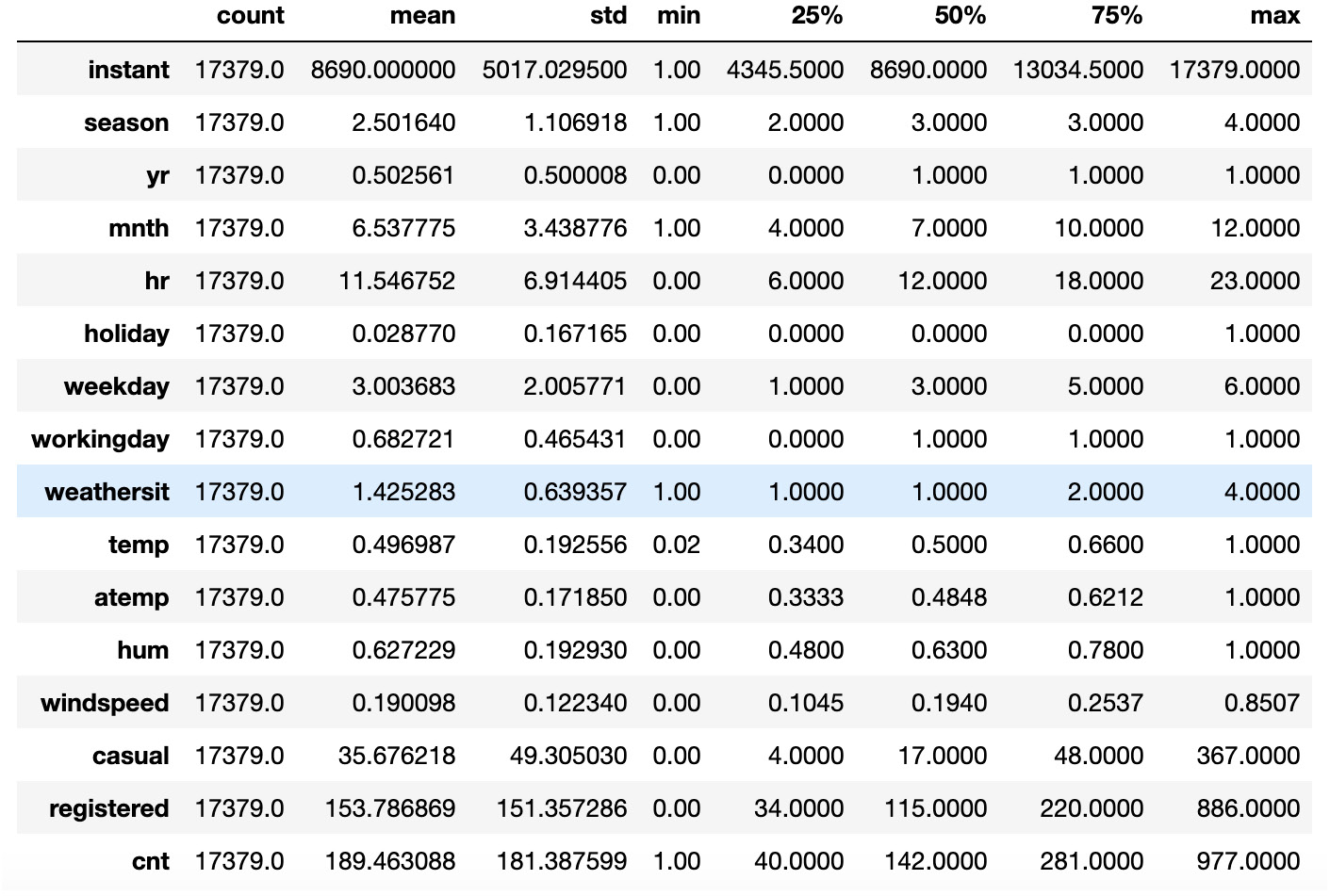Figure 1.1: Output of the describe() method

Note that the `T` character after the `describe()` method gets the transpose of the resulting dataset, hence the columns become rows and vice versa.

According to the description of the original data, provided in the `Readme.txt` file, we can split the columns into three main groups:

• `temporal features`: This contains information about the time at which the record was registered. This group contains the `dteday`, `season`, `yr`, `mnth`, `hr`, `holiday`, `weekday`, and `workingday` columns.
• `weather related features`: This contains information about the weather conditions. The `weathersit`, `temp`, `atemp`, `hum`, and `windspeed` columns are included in this group.
• `record related features`: This contains information about the number of records for the specific hour and date. This group includes the `casual`, `registered`, and `cnt` columns.

Note that we did not include the first column, `instant`, in any of the previously mentioned groups. The reason for this is that it is an index column and will be excluded from our analysis, as it does not contain any relevant information for our analysis.

# Data Preprocessing

In this section, we perform some preprocessing steps, which will allow us to transform the data into a more human-readable format. Note that data preprocessing and wrangling is one of the most important parts of data analysis. In fact, a lot of hidden patterns and relationships might arise when data is transformed in the correct way.

Furthermore, some machine learning algorithms might not even converge, or they may provide an erroneous result when fed with badly preprocessed data (a typical example of this is not scaling data in deep learning). In other cases, deriving insights from normalized data might be difficult for a human; therefore, it is good practice to transform the data before presenting the results.

In this use case, the data is already normalized and ready for analysis; nevertheless, some of its columns are hard to interpret from a human perspective. Before proceeding further, we will perform some basic transformations on the columns, which will result in a more easy-to-understand analysis at a later stage.

## Exercise 1.01: Preprocessing Temporal and Weather Features

In the first part of this exercise, we are going to encode the temporal features into a more human-readable format. The `seasons` column contains values from 1 to 4, which encode, respectively, the `Winter`, `Spring`, `Summer`, and `Fall` seasons. The `yr` column contains the values `0` and `1` representing 2011 and 2012, while the `weekday` column contains values from 0 to 6, with each one representing a day of the week (`0`: `Sunday`, `1`: `Monday`, through to `6`: `Saturday`). Furthermore, we scale the `hum` column to values between 0 and 100 (as it represents the humidity percentage), and the `windspeed` column to values between 0 and 67 (as those are the registered minimum and maximum wind speed):

1. As a first step, create a copy of the original dataset. This is done as we do not want a specific transformation to affect our initial data:
```# create a copy of the original data
preprocessed_data = hourly_data.copy()```
2. In the next step, map the `season` variable from a numerical to a nicely encoded categorical one. In order to do that, we create a Python dictionary, which contains the encoding, and then exploit the `apply` and `lambda` functions:
```# transform seasons
seasons_mapping = {1: 'winter', 2: 'spring', \
3: 'summer', 4: 'fall'}
preprocessed_data['season'] = preprocessed_data['season']\
.apply(lambda x: seasons_mapping[x])```
3. Create a Python dictionary for the `yr` column as well:
```# transform yr
yr_mapping = {0: 2011, 1: 2012}
preprocessed_data['yr'] = preprocessed_data['yr']\
.apply(lambda x: yr_mapping[x])```
4. Create a Python dictionary for the `weekday` column:
```# transform weekday
weekday_mapping = {0: 'Sunday', 1: 'Monday', 2: 'Tuesday', \
3: 'Wednesday', 4: 'Thursday', 5: 'Friday', \
6: 'Saturday'}
preprocessed_data['weekday'] = preprocessed_data['weekday']\
.apply(lambda x: weekday_mapping[x])```

Let's now proceed with encoding the weather-related columns (`weathersit`, `hum`, and `windspeed`). According to the information provided by the data, the `weathersit` column represents the current weather conditions, where `1` stands for clear weather with a few clouds, `2` represents cloudy weather, `3` relates to light snow or rain, and `4` stands for heavy snow or rain. The `hum` column stands for the current normalized air humidity, with values from 0 to 1 (hence, we will multiply the values of this column by 100, in order to obtain percentages). Finally, the `windspeed` column represents the windspeed, which is again normalized to values between 0 and 67 m/s.

5. Encode the `weathersit` values:
```# transform weathersit
weather_mapping = {1: 'clear', 2: 'cloudy', \
3: 'light_rain_snow', 4: 'heavy_rain_snow'}
preprocessed_data['weathersit'] = preprocessed_data['weathersit']\
.apply(lambda x: \
weather_mapping[x])```
6. Finally, rescale the `hum` and `windspeed` columns:
```# transform hum and windspeed
preprocessed_data['hum'] = preprocessed_data['hum']*100
preprocessed_data['windspeed'] = preprocessed_data['windspeed']\
*67```
7. We can visualize the results from our transformation by calling the `sample()` method on the newly created dataset:
```# visualize preprocessed columns
cols = ['season', 'yr', 'weekday', \
'weathersit', 'hum', 'windspeed']
preprocessed_data[cols].sample(10, random_state=123)```

The output should be as follows: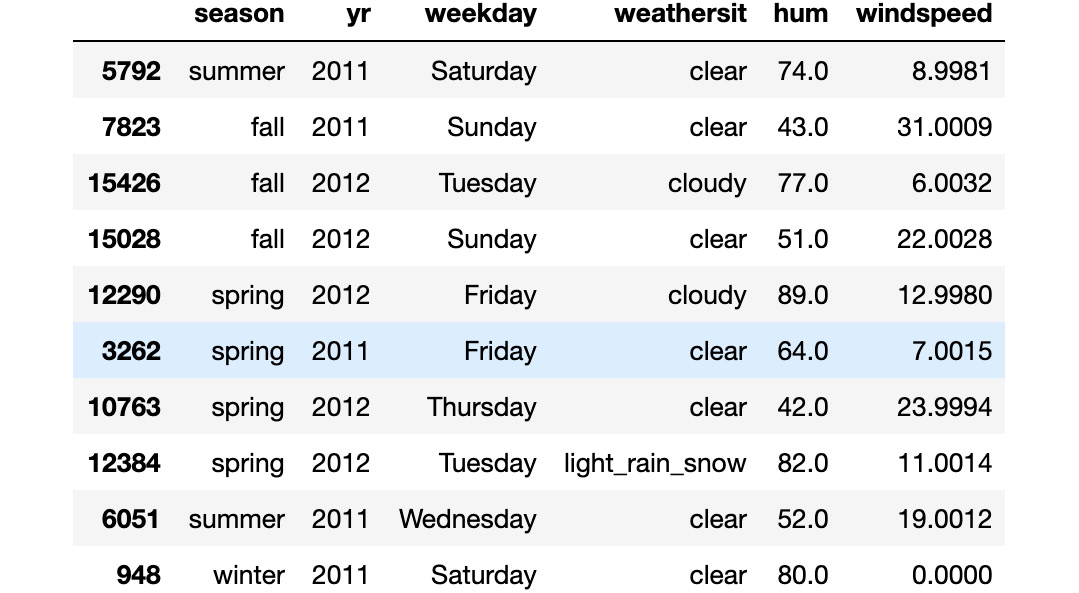Figure 1.2: Result from the transformed variables

Note

To access the source code for this specific section, please refer to https://packt.live/2AFELjq.

You can also run this example online at https://packt.live/3e6xesx. You must execute the entire Notebook in order to get the desired result.

As you can see from the output, the transformed features have categorial values instead of numerical ones. This makes the data more readable and allows certain `pandas` functions to correctly plot the data (as will be demonstrated later).

Note that, in this exercise, we did not transform the `temp` and `atemp` columns (that is, the true and perceived temperatures, respectively). The reason for this is that they assume only positive values in the original dataset (hence, we do not know when the negative temperatures occurred). Furthermore, as their scales are different (the maximum value registered in the true temperature is 41 degrees, while the perceived one is 67), we do not want to modify their relations (that is, the hours at which the true temperature is greater than the perceived one and vice versa).

## Registered versus Casual Use Analysis

We begin our analysis of the single features by focusing on the two main ones: the number of rides performed by registered users versus the number of rides performed by non-registered (or casual) ones. These numbers are represented in the `registered` and `casual` columns, respectively, with the `cnt` column representing the sum of the registered and casual rides. We can easily verify the last statement for each entry in the dataset by using the `assert` statement:

```"""
assert that total number of rides is equal to the sum of registered and casual ones
"""
assert (preprocessed_data.casual \
+ preprocessed_data.registered \
== preprocessed_data.cnt).all(), \
'Sum of casual and registered rides not equal '\
'to total number of rides'```

Note

The triple-quotes ( `"""` ) shown in the code snippet above are used to denote the start and end points of a multi-line code comment. Comments are added into code to help explain specific bits of logic.

The first step in analyzing the two columns is to look at their distributions. A useful Python package that we will use extensively in this book is `seaborn`. It is a data visualization library built on top of the standard `matplotlib` package, which provides a high-level interface for various statistical plots. In this way, the plots we present later will be both nicer and easier to produce. Let's start by visualizing the distribution of the `registered` and `casual` rides:

```# plot distributions of registered vs casual rides
sns.distplot(preprocessed_data['registered'], label='registered')
sns.distplot(preprocessed_data['casual'], label='casual')
plt.legend()
plt.xlabel('rides')
plt.title("Rides distributions")
plt.savefig('figs/rides_distributions.png', format='png')```

The output should be as follows: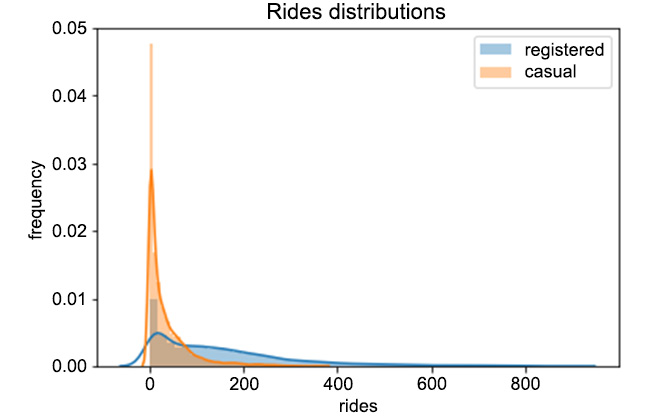Figure 1.3: Distributions of registered versus casual rides per hour

From Figure 1.3, we can easily see that registered users perform way more rides than casual ones. Furthermore, we can see that the two distributions are skewed to the right, meaning that, for most of the entries in the data, zero or a small number of rides were registered (think, for example, of overnight rides). Finally, every entry in the data has quite a large number of rides (that is, higher than 800).

Let's now focus on the evolution of rides over time. We can analyze the number of rides each day with the following piece of code:

```# plot evolution of rides over time
plot_data = preprocessed_data[['registered', 'casual', 'dteday']]
ax = plot_data.groupby('dteday').sum().plot(figsize=(10,6))
ax.set_xlabel("time");
ax.set_ylabel("number of rides per day");
plt.savefig('figs/rides_daily.png', format='png')```

Here, we first take a subset of the original `preprocessed_data` dataset. Afterward, we compute the total number of rides for each day by first grouping the data by the `dteday` column, and then summing the single entries for the `casual` and `registered` columns. The result of the code snippet is given in the following figure: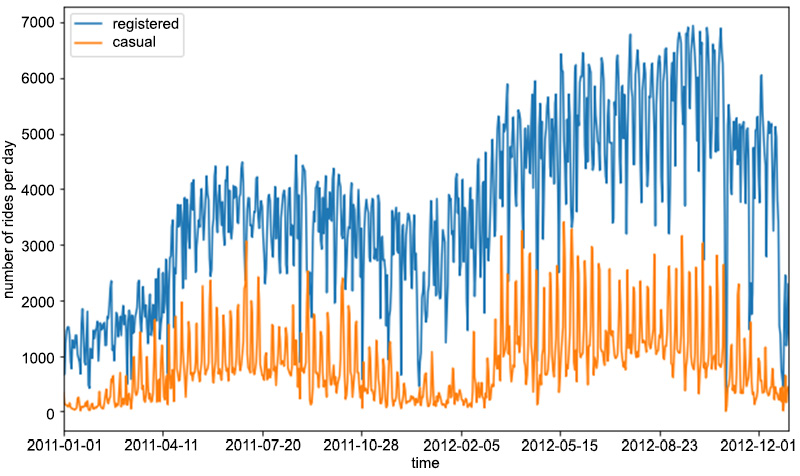Figure 1.4: Evolution of the number of rides per day for registered and casual customers

As you can see from the preceding figure, the number of registered rides is always above and significantly higher than the number of casual rides per day. Furthermore, we can observe that during winter, the overall number of rides decreases (which is totally in line with our expectations, as bad weather and low temperatures have a negative impact on ride sharing services). Note that there is quite a lot of variance in the time series of the rides in Figure 1.4. One way to smooth out the curves is to take the rolling mean and standard deviation of the two time series and plot those instead. In this way, we can visualize not only the average number of rides for a specific time period (also known as a window) but also the expected deviation from the mean:

```"""
Create new dataframe with necessary for plotting columns, and obtain number of rides per day, by grouping over each day
"""
plot_data = preprocessed_data[['registered', 'casual', 'dteday']]
plot_data = plot_data.groupby('dteday').sum()
"""
define window for computing the rolling mean and standard deviation
"""
window = 7
rolling_means = plot_data.rolling(window).mean()
rolling_deviations = plot_data.rolling(window).std()
"""
Create a plot of the series, where we first plot the series of rolling means, then we color the zone between the series of rolling means +- 2 rolling standard deviations
"""
ax = rolling_means.plot(figsize=(10,6))
ax.fill_between(rolling_means.index, rolling_means['registered'] \
+ 2*rolling_deviations['registered'], \
rolling_means['registered'] \
- 2*rolling_deviations['registered'], \
alpha = 0.2)
ax.fill_between(rolling_means.index, rolling_means['casual'] \
+ 2*rolling_deviations['casual'], \
rolling_means['casual'] \
- 2*rolling_deviations['casual'], \
alpha = 0.2)
ax.set_xlabel("time");
ax.set_ylabel("number of rides per day");
plt.savefig('figs/rides_aggregated.png', format='png')```

The preceding code snippet produces the following figure: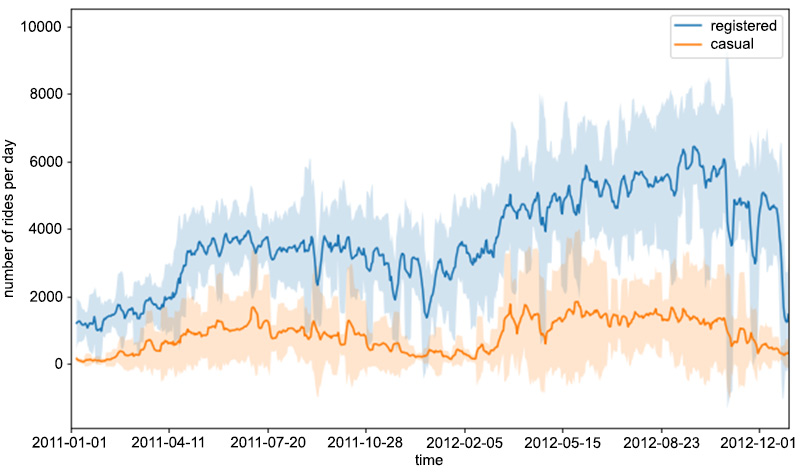Figure 1.5: The rolling mean and standard deviation of rides

In order to compute the rolling statistics (that is, the mean and standard deviation), we use the `rolling()` function, in which we use `mean()` and `std()` to compute the rolling mean and standard deviation, respectively. This is a handy way to compute rolling statistics on time series, in which only recent entries account for computing them. In other words, the value of the rolling mean (or the standard deviation) at a certain time instance is only computed from the last window entries in the time series (in our case, this is 7), and not from the entries of the whole series.

Let's now focus on the distributions of the requests over separate hours and days of the week. We would expect certain time patterns to arise, as bike requests should be more frequent during certain hours of the day, depending on the day of the week. This analysis can be easily done by leveraging various functions from the `seaborn` package, as shown in the following code snippet:

```# select relevant columns
plot_data = preprocessed_data[['hr', 'weekday', 'registered', 'casual']]
"""
transform the data into a format, in number of entries are computed as count,
for each distinct hr, weekday and type (registered or casual)
"""
plot_data = plot_data.melt(id_vars=['hr', 'weekday'], \
var_name='type', value_name='count')
"""
create FacetGrid object, in which a grid plot is produced.
As columns, we have the various days of the week,
as rows, the different types (registered and casual)
"""
grid = sns.FacetGrid(plot_data, row='weekday', \
col='type', height=2.5, aspect=2.5, \
row_order=['Monday', 'Tuesday', \
'Wednesday', 'Thursday', \
'Friday', 'Saturday', 'Sunday'])
# populate the FacetGrid with the specific plots
grid.map(sns.barplot, 'hr', 'count', alpha=0.5)
grid.savefig('figs/weekday_hour_distributions.png', format='png')```

Let's focus on the `melt()` function, applied on a `pandas` dataset. It will create a new dataset, in which values are grouped by the `hr` and `weekday` columns, while creating two new columns: `type` (containing the `casual` and `registered` values) and `count` (containing the respective counts for the `casual` and `registered` types).

The `seaborn.FacetGrid()` function will create a new grid of plots, with rows corresponding to the different days of the week and columns corresponding to the types. Finally, the `map()` function is applied to each element of the grid, creating the respective plots. The produced plot is shown in Figure 1.6. We can immediately note that on working days, the highest number of rides for registered users takes place around 8 AM and at 6 PM. This is totally in line with our expectations, as it is likely that most registered users use the bike sharing service for commuting. On the other hand, the casual usage of bike sharing services on working days is quite limited, as the plot shows.

During the weekend, we can see that ride distributions change for both casual and registered users. Still, registered rides are more frequent than casual ones, but both the distributions have the same shape, almost uniformly distributed between the time interval of 11 AM to 6 PM.

As a conclusion, we could claim that most of the usage of bike sharing services occurs during working days, right before and right after the standard working time (that is, 9 to 5):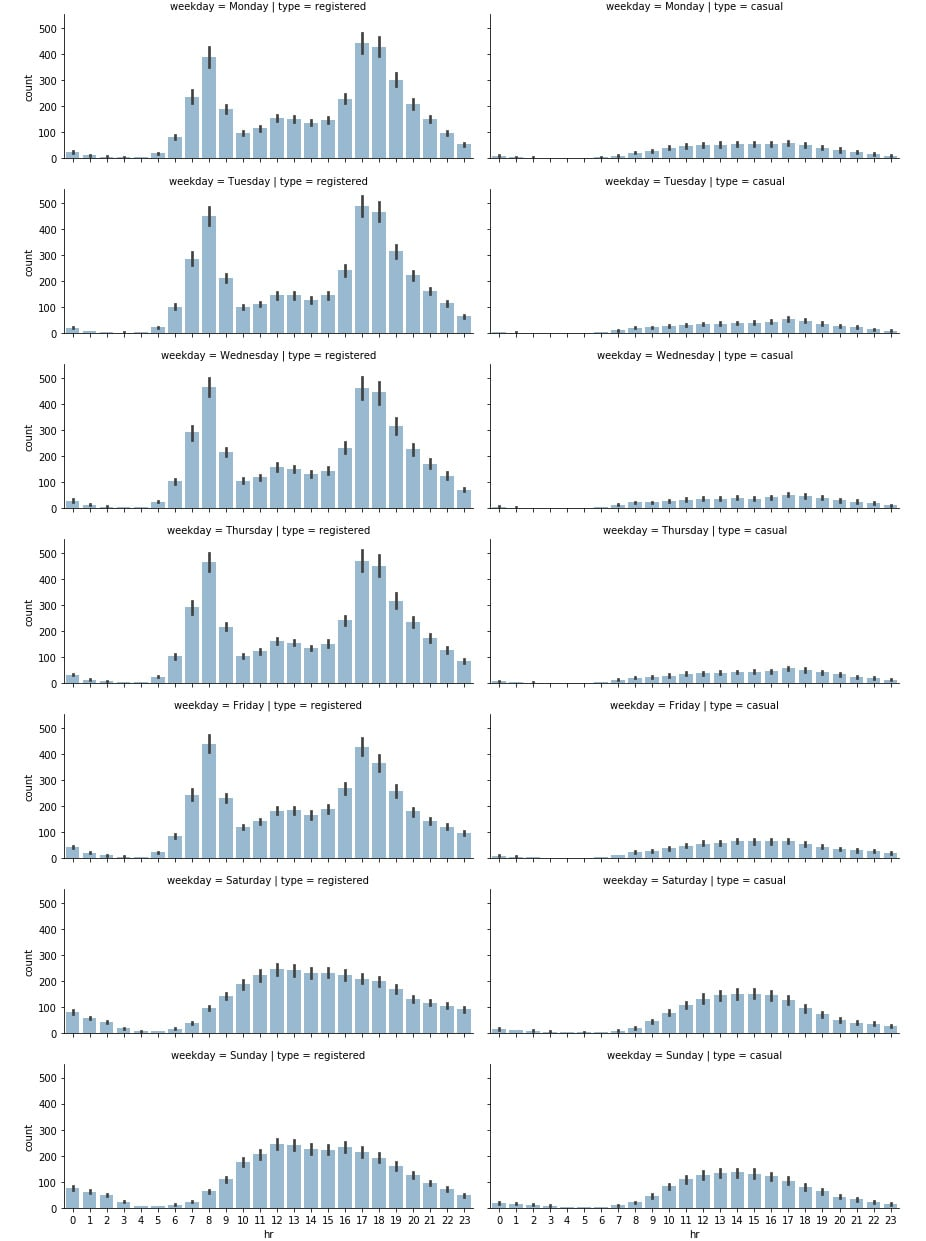Figure 1.6: Distribution of rides on a daily and hourly basis

## Exercise 1.02: Analyzing Seasonal Impact on Rides

In this exercise, we will investigate the impact of the different seasons on the total number of rides. Our goal is to create grid plots, similar to the one in Figure 1.6, in which the number of rides will be distributed over hours and weekdays, based on the current season. This exercise is a continuation of Exercise 1.01, Preprocessing Temporal and Weather Features:

1. Start by combining the hours and seasons. Create a subset of the initial data by selecting the `hr`, `season`, `registered`, and `casual` columns:
```# select subset of the data
plot_data = preprocessed_data[['hr', 'season', \
'registered', 'casual']]```
2. Next, unpivot the data from wide to long format:
```# unpivot data from wide to long format
plot_data = plot_data.melt(id_vars=['hr', 'season'], \
var_name='type', value_name='count')```
3. Define the seaborn `FacetGrid` object, in which rows represent the different seasons:
```# define FacetGrid
grid = sns.FacetGrid(plot_data, row='season', \
col='type', height=2.5, \
aspect=2.5, \
row_order=['winter', 'spring', \
'summer', 'fall'])```

Note that we are also specifying the desired order of rows here. We do this as we want the rows to appear in a certain order.

4. Finally, apply the `seaborn.barplot()` function to each of the `FacetGrid` elements:
```# apply plotting function to each element in the grid
grid.map(sns.barplot, 'hr', 'count', alpha=0.5)
# save figure
grid.savefig('figs/exercise_1_02_a.png', format='png')```

The resulting plot is shown in Figure 1.7: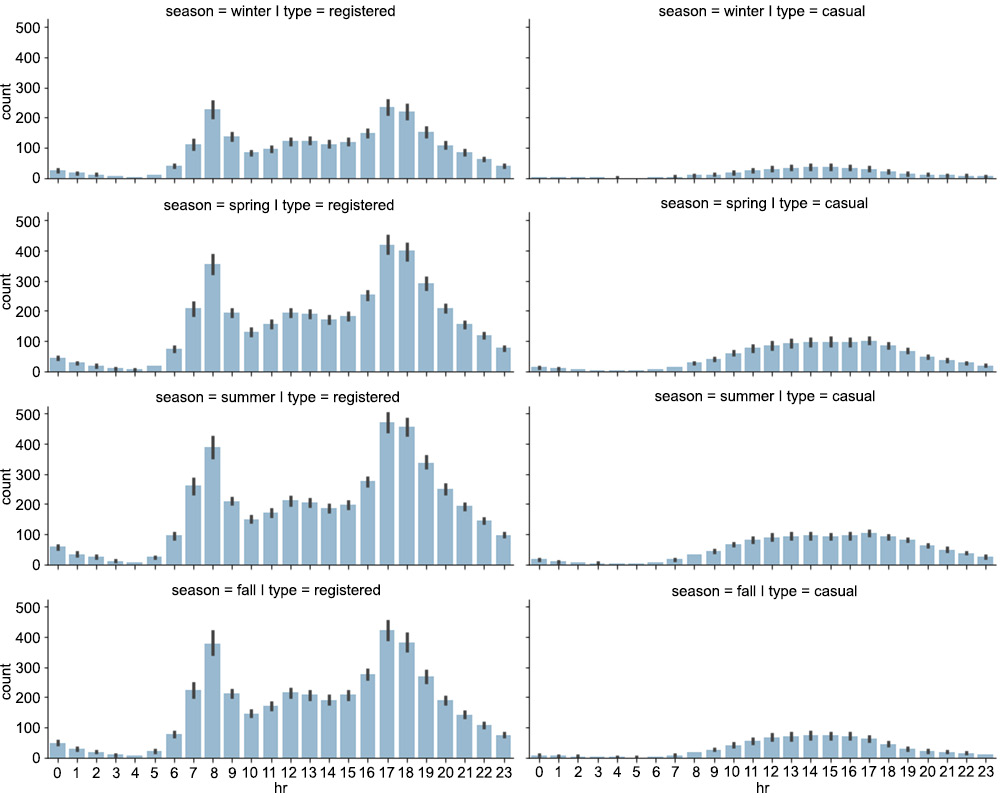Figure 1.7: The distribution of rides on a seasonal level

As can be seen in the plot, while each season has a similar graph shape, the count is lower for the winter graph. So there are fewer rides (registered and casual) during winter. This makes sense, as fewer rides are likely to occur when the weather conditions are poor.

For the second part of the exercise (the distribution of rides on a weekday basis), we proceed just as we did in the first part.

5. First, create a subset of the initial preprocessed data, containing only the relevant columns (`weekday`, `season`, `registered`, and `casual`):
```plot_data = preprocessed_data[['weekday', 'season', \
'registered', 'casual']]```
6. Again unpivot the data from wide to long format, but this time use `weekday` and `season` as grouping variables:
```plot_data = plot_data.melt(id_vars=['weekday', 'season'], \
var_name='type', value_name='count')```
7. The `FacetGrid` object is created using the `seaborn.FacetGrid()` function:
```grid = sns.FacetGrid(plot_data, row='season', col='type', \
height=2.5, aspect=2.5, \
row_order=['winter', 'spring', \
'summer', 'fall'])```
8. Finally, apply the `seaborn.barplot()` function to each of the elements in the `FacetGrid` object:
```grid.map(sns.barplot, 'weekday', 'count', alpha=0.5, \
order=['Monday', 'Tuesday', 'Wednesday', 'Thursday', \
'Friday', 'Saturday', 'Sunday'])```

Note that we are also specifying the order of the days of the week, which is passed as a parameter to the `seaborn.barplot()` function. The resulting plot is shown in the following figure: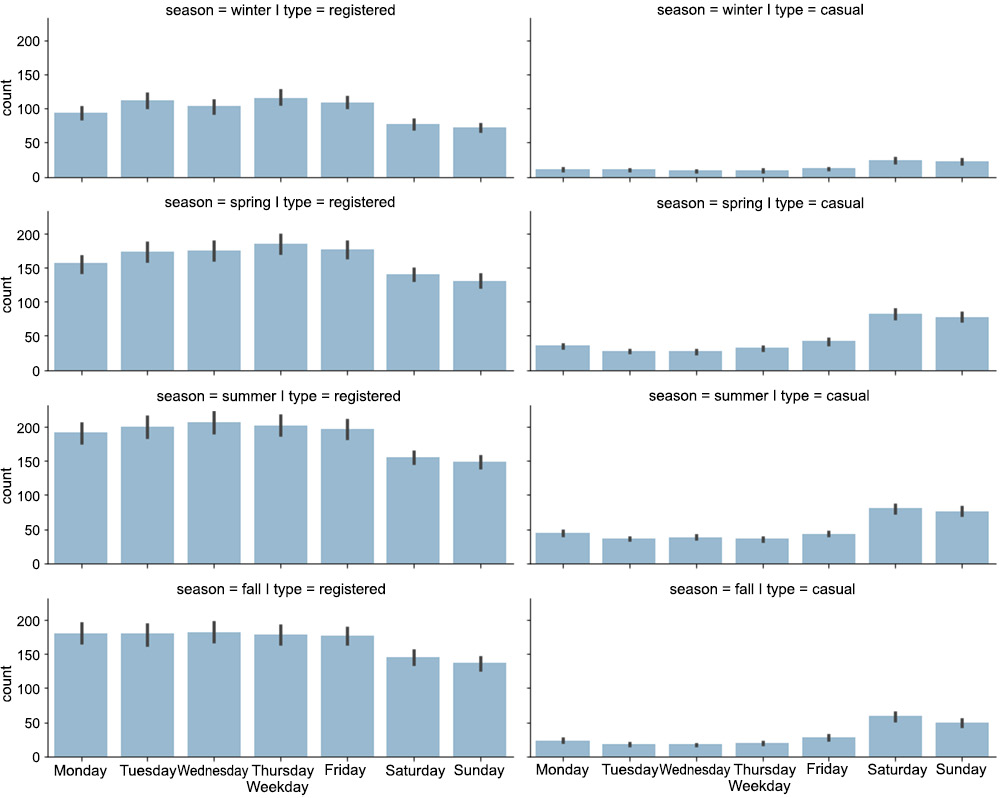Figure 1.8: The distribution of rides over the days of the week

Note

To access the source code for this specific section, please refer to https://packt.live/2Y43Kpx.

You can also run this example online at https://packt.live/30hgeda. You must execute the entire Notebook in order to get the desired result.

An interesting pattern occurs from the analysis conducted in Exercise 1.02, Analyzing Seasonal Impact on Rides. There is a decreasing number of registered rides over the weekend (compared to the rest of the week), while the number of casual rides increases. This could enforce our initial hypothesis, that is, that registered customers mostly use the bike sharing service for commuting (which could be the reason for the decreasing number of registered rides over the weekend), while casual customers use the service occasionally over the weekend. Of course, such a conclusion cannot be based solely on plot observations but has to be backed by statistical tests, which is the topic of our next section.

# Hypothesis Tests

Hypothesis testing is a branch of inferential statistics, that is, a part of the statistics field in which a general conclusion can be done about a large group (a population) based on the analysis and measurements performed on a smaller group (a sample). A typical example could be making an estimation of the average height of a country's citizens (in this case, the population) based on measurements performed on a thousand people (the sample). Hypothesis testing tries to address the question, "Is a certain hypothetical value in line with the value obtained by direct measurements or not?"

Although various statistical tests are known in the literature and in practice, the general idea can be summarized in the following steps:

• Definition of null and alternative hypotheses: In this first step, a null hypothesis (denoted as H0) is defined (let's say H0 is 'the average country's population height is 175 cm'). This is the hypothesis that is going to be tested by the statistical test. The alternative hypothesis (denoted as Ha) consists of the complement statement of the null hypothesis (in our example, the alternative hypothesis, Ha, is 'the average height is not 175 cm'). The null and alternative hypotheses always complement one another.
• Identifying the appropriate test statistic: A test statistic is a quantity whose calculation is based on the sample, and whose value is the basis for accepting or rejecting the null hypothesis. In most of these cases, it can be computed by the following formula:Figure 1.9: The formula for the test statistic

Here, the sample statistic is the statistic value computed on the sample (in our case, the average height of a thousand people); the value under null hypothesis is the value, assuming that the null hypothesis holds (in this case, 175 cm); and the standard error of the sample statistic is the standard error in the measurement of the sample. Once the test statistic is identified and computed, we have to decide what type of probability distribution it follows. In most of the cases, the following probability distributions will be used: Student's t-distribution (for t-tests); Standard normal or z-distribution (for z-tests); Chi-squared distribution (for a chi-squared test) and F-distribution (for F-tests).

Note

Because exploring probability distributions in more detail is beyond the scope of this book, we refer the reader to the excellent book by Charles Grinstead and Laurie Snell, Introduction to Probability. Take a look at the following link: https://math.dartmouth.edu/~prob/prob/prob.pdf.

Choosing which distribution to use depends on the sample size and the type of test. As a rule of thumb, if the sample size is greater than 30, we can expect that the assumptions of the central limit theorem hold and that the test statistic follows a normal distribution (hence, use a z-test). For a more conservative approach, or for samples with less than 30 entries, a t-test should be used (with a test statistic following Student's t-distribution).

• Specifying the significance level: Once the test statistic has been calculated, we have to decide whether we can reject the null hypothesis or not. In order to do that, we specify a significance level, which is the probability of rejecting a true null hypothesis. A general approach is to specify a level of significance of 5%. This means that we accept that there is a 5% probability that we reject the null hypothesis while being true (for a more conservative approach, we could always use 1% or even 0.5%). Once a significance level is specified, we have to compute the rejection points, which are the values with which the test statistic is compared. If it is larger than the specified rejection point(s), we can reject the null hypothesis and assume that the alternative hypothesis is true. We can distinguish two separate cases here.
• Two-sided tests: These are tests in which the null hypothesis assumes that the value "is equal to" a predefined value. For example, the average height of the population is equal to 175 cm. In this case, if we specify a significance level of 5%, then we have two critical values (one positive and one negative), with the probability of the two tails summing up to 5%. In order to compute the critical values, we have to find the two percentiles of a normal distribution, such that the probability within those two values is equal to 1 minus the significance level. For example, if we assume that the sample mean of the height follows a normal distribution, with a level of significance for our test of 5%, then we need to find the two percentiles, with the probability that a value drawn from a normal distribution falls outside of those values, equal to 0.05. As the probability is split between the two tails, the percentiles that we are looking at are the 2.5 and 97.5 percentiles, corresponding to the values -1.96 and 1.96 for a normal distribution. Hence, we will not reject the null hypothesis if the following holds true: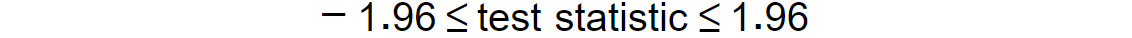Figure 1.10: Test statistic limit for a two-sided test

If the preceding formula does not hold true, that is, the `test statistic` is greater than `1.96` or less than -1.96, we will reject the null hypothesis.

• One-sided tests: These are tests in which the null hypothesis assumes that the value is "greater than" or "less than" a predefined value (for example, the average height is greater than 175 cm). In that case, if we specify a significance level of 5%, we will have only one critical value, with a probability at the tail equal to 5%. In order to find the critical value, we have to find the percentile of a normal distribution, corresponding to a probability of `0.05` at the tail. For tests of the "greater than" type, the critical value will correspond to the 5-th percentile, or `-1.645` (for tests following a normal distribution), while for tests of the "less than" type, the critical value will correspond to the 95-th percentile, or 1.645. In this way, we will reject the null hypothesis for tests "greater than" if the following holds true: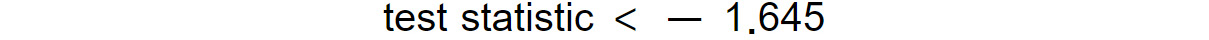Figure 1.11: Test statistic limit for a one-sided test

Whereas, for tests of the "less than" type, we reject the null hypothesis if the following is the case: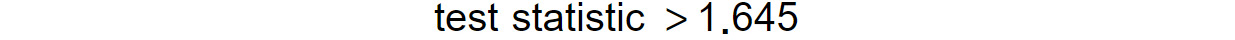Figure 1.12: Test statistic limit for a one-sided test

Note that, quite often, instead of computing the critical values of a certain significance level, we refer to the p-value of the test. The p-value is the smallest level of significance at which the null hypothesis can be rejected. The p-value also provides the probability of obtaining the observed sample statistic, assuming that the null hypothesis is correct. If the obtained p-value is less than the specified significance level, we can reject the null hypothesis, hence the p-value approach is, in practice, an alternative (and, most of the time, a more convenient) way to perform hypothesis testing.

Let's now provide a practical example of performing hypothesis testing with Python.

## Exercise 1.03: Estimating Average Registered Rides

In this exercise, we will show how to perform hypothesis testing on our bike sharing dataset. This exercise is a continuation of Exercise 1.02, Analyzing Seasonal Impact on Rides:

1. Start with computing the average number of registered rides per hour. Note that this value will serve in formulating the null hypothesis because, here, you are explicitly computing the population statistic—that is, the average number of rides. In most of the cases, such quantities are not directly observable and, in general, you only have an estimation for the population statistics:
```# compute population mean of registered rides
population_mean = preprocessed_data.registered.mean()```
2. Suppose now that you perform certain measurements, trying to estimate the true average number of rides performed by registered users. For example, register all the rides during the summer of 2011 (this is going to be your sample):
```# get sample of the data (summer 2011)
sample = preprocessed_data[(preprocessed_data.season \
== "summer") \
& (preprocessed_data.yr \
== 2011)].registered```
3. Specify the significance level. A standard value is 0.05 (that is, when performing the statistical test), if the p-value obtained by the statistical test is less than 0.05, you can reject the null hypothesis by at least 95%. The following code snippet shows you how to do that:
```# perform t-test and compute p-value
from scipy.stats import ttest_1samp
test_result = ttest_1samp(sample, population_mean)
print(f"Test statistic: {test_result}, \
p-value: {test_result}")```

The output should be as follows:

`Test statistic: -3.492, p-value: 0.000`

The result of the previous test returns a p-value smaller than 0.001, which is less than the predefined critical value. Therefore, you can reject the null hypothesis and assume that the alternative hypothesis is correct.

Note that you have to make an important observation here: You computed the average number of rides on the true population; therefore, the value computed by the statistical test should be the same. So why have you rejected the null hypothesis? The answer to that question lies in the fact that your sample is not a true representation of the population, but rather a biased one. In fact, you selected only entries from the summer of 2011. Therefore, neither data from the full year is present, nor entries from 2012.

4. In order to show how such mistakes can compromise the results of statistical tests, perform the test again, but this time taking as a sample 5% of the registered rides (selected randomly). The following code snippet performs that:
```# get sample as 5% of the full data
import random
random.seed(111)
sample_unbiased = preprocessed_data.registered.sample(frac=0.05)
test_result_unbiased = ttest_1samp(sample_unbiased, \
population_mean)
print(f"Unbiased test statistic: {test_result_unbiased}, \
p-value: {test_result_unbiased}")```

The output should be as follows:

`Unbiased test statistic: -2.008, p-value: 0.045`

This time, the computed p-value is equal to 0.45, which is much larger than the critical 0.05, and so, you cannot reject the null hypothesis.

Note

To access the source code for this specific section, please refer to https://packt.live/2N3W1la.

You can also run this example online at https://packt.live/3fxDYjt. You must execute the entire Notebook in order to get the desired result.

In this exercise, we performed hypothesis testing with Python on the bike sharing dataset. Furthermore, we saw the importance of having an unbiased sample of the data, as test results can be easily compromised if working with biased data.

Quite often, when performing statistical tests, we want to compare certain statistics on two different groups (for example, the average height between women and men) and estimate whether there is a statistically significant difference between the values obtained in the two groups. Let's denote, with `μ`1 and `μ`2, the hypothetical means of the two groups, where we will have:

• A null hypothesis: `H`0`:μ`1`-μ`2` ≠ 0`
• An alternative hypothesis: `H`a`:μ`1`-μ`2` ≠ 0`

Let's denote, with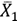and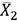, the sample means (that is, the means obtained from the two groups), where the test statistic takes the following form:Figure 1.13: Test statistic with the sample means

Here, `n`1 and `n`2 are the number of samples in the two groups, while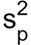is the pooled estimator of the common variance, computed as follows:Figure 1.14: Pooled estimator of the common variance

Here,and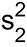are the variances of the two groups. Note that the test statistic, in this case, follows Student's t-distribution with `n`1`+n`2`-2` degrees of freedom.

As in the previous case, most of the time, we don't have to compute the test statistics by ourselves, as Python already provides handy functions for that, plus the alternative approach of accepting or rejecting the null hypothesis using the p-value is still valid.

Let's now focus on a practical example of how to perform a statistical test between two different groups. In the previous section, we observed, graphically, that registered users tend to perform more rides during working days than the weekend. In order to assess this statement, we will perform a hypothesis test in which we will test whether the mean of registered rides during working days is the same as during the weekend. This is done in the following exercise.

## Exercise 1.04: Hypothesis Testing on Registered Rides

In this exercise, we will be performing a hypothesis on registered rides. This exercise is a continuation of Exercise 1.03, Estimating Average Registered Rides:

1. First, formulate the null hypothesis. As mentioned earlier, you are interested in identifying whether there is a statistically significant difference between registered rides during working days and the weekend. Therefore, our null hypothesis is that the average number of rides for registered users during working days is the same as the average number of rides during the weekend. In other words:

`H_0` : average registered rides over weekdays-average registered rides over weekend=0

and

`H_a` : average registered rides over weekdays-average registered rides over weekend≠0

2. Once the null hypothesis is established, collect data for the two groups. This is done with the following code snippet:
```# define mask, indicating if the day is weekend or work day
weekend_days = ['Saturday', 'Sunday']
# select registered rides for the weekend and working days
3. Perform the two-sample t-tests by using the `scipy.stats.ttest_ind` function:
```# perform ttest
from scipy.stats import ttest_ind
test_res = ttest_ind(weekend_data, workingdays_data)
print(f"Statistic value: {test_res:.03f}, \
p-value: {test_res:.03f}")```

The output should be as follows:

`Statistic value: -16.004, p-value: 0.000`

The resulting p-value from this test is less than 0.0001, which is far below the standard critical 0.05 value. As a conclusion, we can reject the null hypothesis and confirm that our initial observation is correct: that is, there is a statistically significant difference between the number of rides performed during working days and the weekend.

4. Plot the distributions of the two samples:
```"""
plot distributions of registered rides for working vs weekend days
"""
sns.distplot(weekend_data, label='weekend days')
sns.distplot(workingdays_data, label='working days')
plt.legend()
plt.xlabel('rides')
plt.ylabel('frequency')
plt.title("Registered rides distributions")
plt.savefig('figs/exercise_1_04_a.png', format='png')```

The output should be as follows: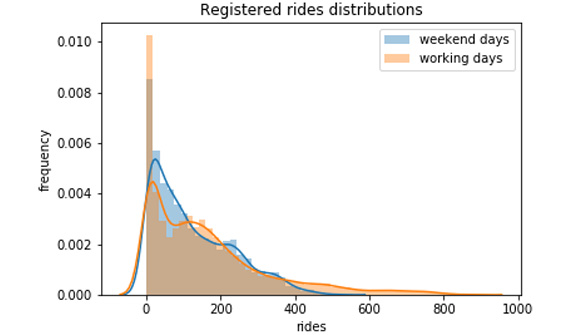Figure 1.15: The distribution of registered rides: working days versus the weekend

5. Perform the same type of hypothesis testing to validate the second assumption from the last section— that is, casual users perform more rides during the weekend. In this case, the null hypothesis is that the average number of rides during working days is the same as the average number of rides during the weekend, both performed only by casual customers. The alternative hypothesis will then result in a statistically significant difference in the average number of rides between the two groups:
```# select casual rides for the weekend and working days
# perform ttest
test_res = ttest_ind(weekend_data, workingdays_data)
print(f"Statistic value: {test_res:.03f}, \
p-value: {test_res:.03f}")
# plot distributions of casual rides for working vs weekend days
sns.distplot(weekend_data, label='weekend days')
sns.distplot(workingdays_data, label='working days')
plt.legend()
plt.xlabel('rides')
plt.ylabel('frequency')
plt.title("Casual rides distributions")
plt.savefig('figs/exercise_1_04_b.png', format='png')```

The output should be as follows:

`Statistic value: 41.077, p-value: 0.000`

The p-value returned from the previous code snippet is 0, which is strong evidence against the null hypothesis. Hence, we can conclude that casual customers also behave differently over the weekend (in this case, they tend to use the bike sharing service more) as seen in the following figure: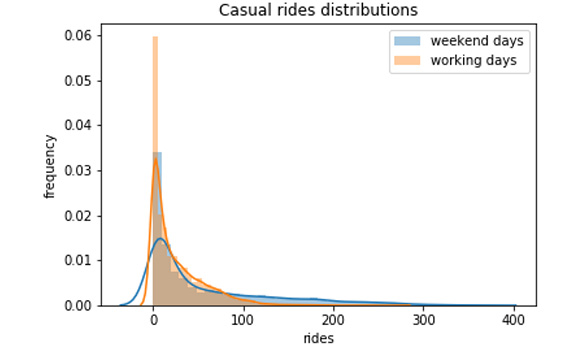Figure 1.16: Distribution of casual rides: working days versus the weekend

Note

To access the source code for this specific section, please refer to https://packt.live/3fxe7rY.

You can also run this example online at https://packt.live/2C9V0pf. You must execute the entire Notebook in order to get the desired result.

In conclusion, we can say that there is a statistically significant difference between the number of rides on working days and weekend days for both casual and registered customers.

# Analysis of Weather-Related Features

Let's now focus on an analysis of the group of features representing the weather conditions. Our expectation is to observe a strong dependency of those features on the current number of rides, as bad weather can significantly influence bike sharing services.

The weather features we identified earlier are the following:

• `weathersit`: This is a categorical variable representing the current weather situation. We encoded this variable with the following four values: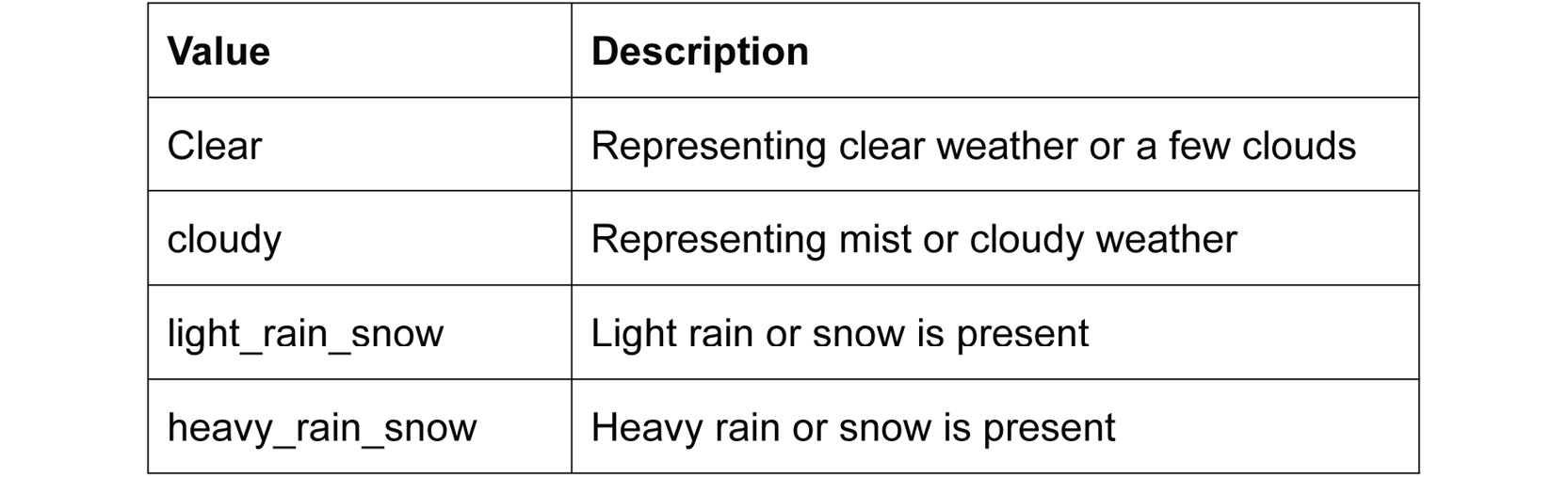Figure 1.17: Description of weather features

• `temp`: This is the normalized temperature in Celsius. Values are divided by 41, which means that the highest registered temperature in the data is 41°C (corresponding to 1 in our dataset).
• `atemp`: The normalized feeling temperature in Celsius. Values are divided by 50, which means that the highest registered temperature in the data is 50°C (corresponding to 1 in our dataset).
• `hum`: The humidity level as a percentage.
• `windspeed`: The wind speed in m/s.

From the provided descriptions, we can see that most of the weather-related features assume continuous values (except for `weathersit`). Furthermore, as both our variables of interest (the `casual` and `registered` number of rides) are also continuously distributed, the first and most common way to measure the relationship between two different continuous variables is to measure their correlation.

Correlation (also known as Pearson's correlation) is a statistic that measures the degree to which two random variables move in relation to each other. In practice, it provides a numerical measure (scaled between -1 and 1), through which we can identify how much one of the variables would move in one direction, assuming that the other one moves. Let's denote, with `X` and `Y`, the two random variables. The correlation coefficient between `X` and `Y` is denoted with `ρ(X,Y)` and is computed by the formula:Figure 1.18: The correlation coefficient between X and Y

Here,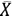and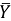denote the mean of the two variables, and `X`i and `Y`i represent the individual data points in set `X` and set `Y`. A positive correlation between `X` and `Y` means that increasing one of the values will increase also the other one, while a negative correlation means that increasing one of the values will decrease the other one.

Let's provide a practical example on computing the correlation between two variables. As we want to compare several variables, it makes sense to define a function that performs the analysis between the variables, as we want to follow the Don't Repeat Yourself principle (commonly known as DRY):

```def plot_correlations(data, col):
# get correlation between col and registered rides
corr_r = np.corrcoef(data[col], data["registered"])[0,1]
ax = sns.regplot(x=col, y="registered", data=data, \
scatter_kws={"alpha":0.05}, \
label=f"Registered rides \
(correlation: {corr_r:.3f})")
# get correlation between col and casual rides
corr_c = np.corrcoef(data[col], data["casual"])[0,1]
ax = sns.regplot(x=col, y='casual', data=data, \
scatter_kws={"alpha":0.05}, \
label=f"Casual rides (correlation: {corr_c:.3f})")
legend = ax.legend()
for lh in legend.legendHandles:
lh.set_alpha(0.5)
ax.set_ylabel("rides")
ax.set_title(f"Correlation between rides and {col}")
return ax```

Applying the previously defined function to the four columns (`temp`, `atemp`, `hum`, and `windspeed`) returns the following figure:

```plt.figure(figsize=(10,8))
ax = plot_correlations(preprocessed_data, 'temp')
plt.savefig('figs/correlation_temp.png', format='png')
plt.figure(figsize=(10,8))
ax = plot_correlations(preprocessed_data, 'atemp')
plt.savefig('figs/correlation_atemp.png', format='png')```

The output should be as follows: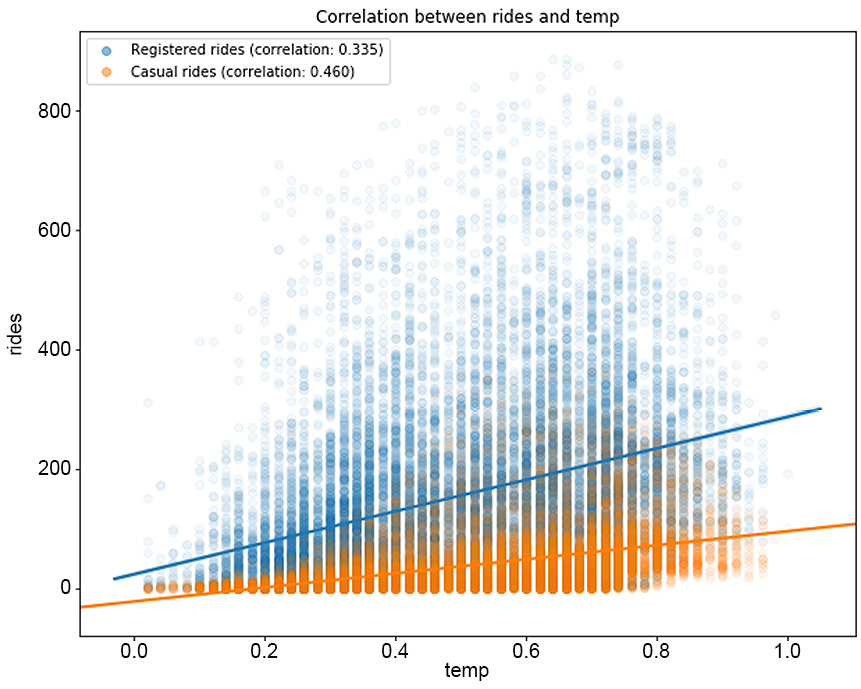Figure 1.19: The correlation between rides and temp

The plot for correlation between rides and `atemp` would be as follows: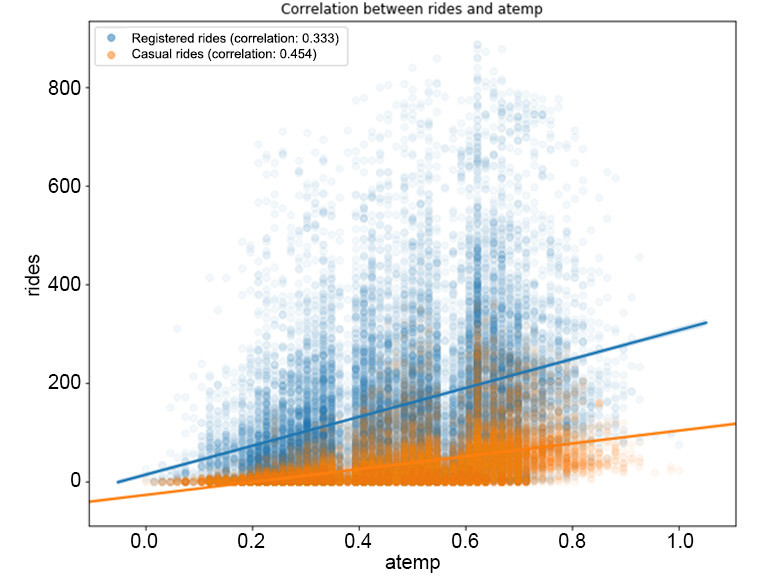Figure 1.20: The correlation between the rides and atemp features

Now plot the correlation between the rides and `hum`, `windspeed` features separately:

```plt.figure(figsize=(10,8))
ax = plot_correlations(preprocessed_data, 'hum')
plt.savefig('figs/correlation_hum.png', format='png')
plt.figure(figsize=(10,8))
ax = plot_correlations(preprocessed_data, 'windspeed')
plt.savefig('figs/correlation_windspeed.png', format='png')```

The output should be as follows: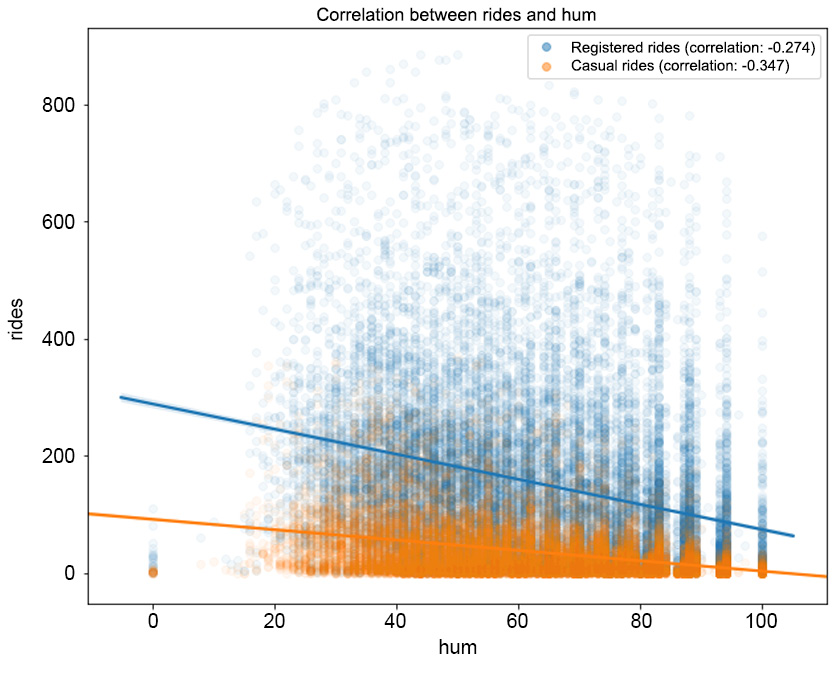Figure 1.21: The correlation between rides and hum

The correlation between rides and `windspeed` can be visualized as follows: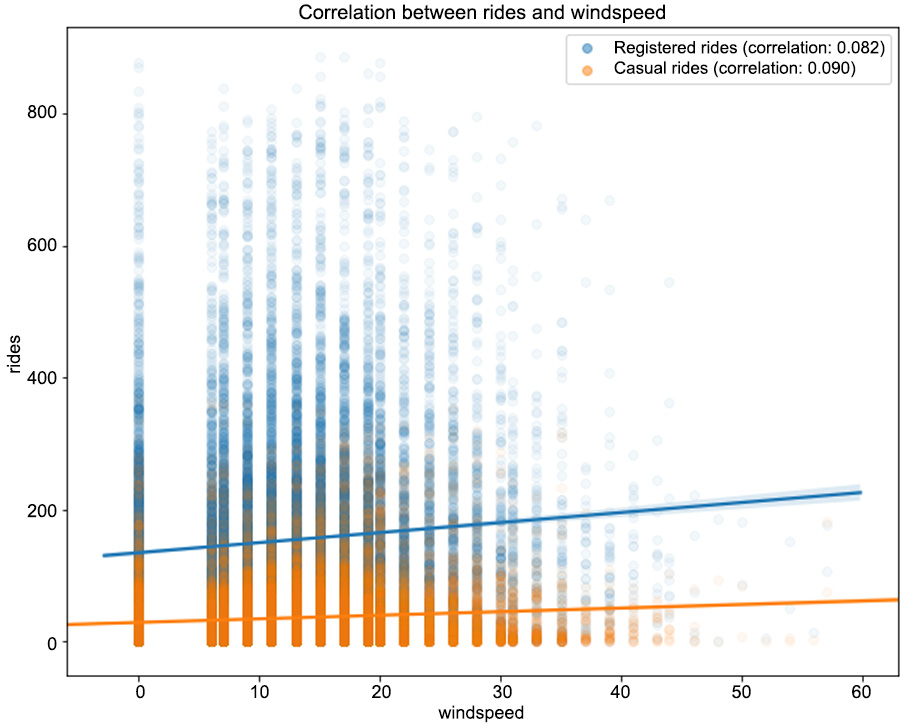Figure 1.22: The correlation between the rides and windspeed features

From Figure 1.19, we can observe that higher temperatures have a positive impact on the number of rides (the correlation between registered/casual rides and `temp` is 0.335 and 0.46, respectively, and it's a similar case for `atemp`). Note that as the values in the `registered` column are widely spread with respect to the different values in `temp`, we have a lower correlation compared to the `casual` column. The same pattern can be observed in Figure 1.21, in which the humidity level has a negative correlation with both types of rides (-0.274 for `registered` and -0.347 for `casual`). This means that with a high level of humidity (mist or rain), customers will tend not to use the bike sharing service. From Figure 1.22, we can see that there is minimal correlation between the number of rides and the wind speed (a weak positive correlation).

One of the major drawbacks of the correlation coefficient is its assumption of a linear relationship between the two random variables. This is quite a strong assumption as, most of the time, relationships in nature are not linear. A measure that generalizes the Pearson's correlation to monotonic relationships between two variables is the Spearman rank correlation.

Let's illustrate the difference between the two measures in the following example.

## Exercise 1.05: Evaluating the Difference between the Pearson and Spearman Correlations

In this exercise, you will investigate the difference between the Pearson correlation (in which a linear relationship between the two variables is assumed) and the Spearman correlation (in which only a monotonic relationship is required). This will help you to understand the difference between the two types of correlations, especially when the data does not satisfy the linear assumption. To better present the difference between the two measures, you will create synthetic data that will serve your purpose:

1. Start by defining your random variables. Create an `X` variable, which will represent your independent variable, and two dependent ones, `Y`lin and `Y`mon, which can be expressed as follows: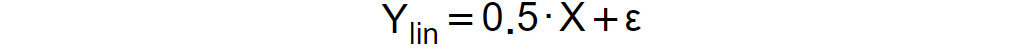Figure 1.23: Expression for the dependent variable YlinFigure 1.24: Expression for the dependent variable Ymon

Here, `ε` represents a noise component, which is normally distributed with a mean of 0 and a standard deviation of 0.1:

```# define random variables
x = np.linspace(0,5, 100)
y_lin = 0.5*x + 0.1*np.random.randn(100)
y_mon = np.exp(x) + 0.1*np.random.randn(100)```
2. Compute the Pearson and Spearman correlations using the `pearsonr()` and `spearmanr()` functions in the `scipy.stats` module:
```# compute correlations
from scipy.stats import pearsonr, spearmanr
corr_lin_pearson = pearsonr(x, y_lin)
corr_lin_spearman = spearmanr(x, y_lin)
corr_mon_pearson = pearsonr(x, y_mon)
corr_mon_spearman = spearmanr(x, y_mon)```

Note that both the `pearsonr()` and `spearmanr()` functions return a two-dimensional array in which the first value is the respective correlation, while the second one is the p-value of a hypothesis test in which the null hypothesis assumes that the computed correlation is equal to zero. This is quite handy at times, as you not only compute the correlation, but also test its statistical significance against being zero.

3. Visualize both the data and the computed correlations:
```# visualize variables
fig, (ax1, ax2) = plt.subplots(1, 2, figsize=(10,5))
ax1.scatter(x, y_lin)
ax1.set_title(f"Linear relationship\n \
Pearson: {corr_lin_pearson:.3f}, \
Spearman: {corr_lin_spearman:.3f}")
ax2.scatter(x, y_mon)
ax2.set_title(f"Monotonic relationship\n \
Pearson: {corr_mon_pearson:.3f}, \
Spearman: {corr_mon_spearman:.3f}")
fig.savefig('figs/exercise_1_05.png', format='png')```

The output should be as follows: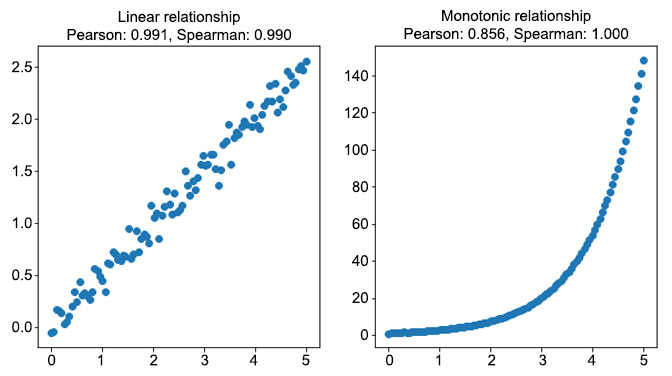Figure 1.25: The difference between the Pearson and Spearman correlations

As you can see from the preceding figure, when the relationship between the two variables is linear (the figure on the left), the two correlation coefficients are very similar. In the monotonic relationship (the figure on the right), the linear assumption of the Pearson correlation fails, and, although the correlation coefficient is still quite high (0.856), it is not capable of capturing the perfect relationship between the two variables. On the other hand, the Spearman correlation coefficient is 1, which means that it succeeds in capturing the almost perfect relationship between the two variables.

4. Now return to the bike sharing data and investigate the relationship between the different variables in light of the difference between the two correlation measures. Define a function that, on the provided data and column, computes the Pearson and Spearman correlation coefficients with the `registered` and `casual` rides:
```# define function for computing correlations
def compute_correlations(data, col):
pearson_reg = pearsonr(data[col], data["registered"])
pearson_cas = pearsonr(data[col], data["casual"])
spearman_reg = spearmanr(data[col], data["registered"])
spearman_cas = spearmanr(data[col], data["casual"])
return pd.Series({"Pearson (registered)": pearson_reg,\
"Spearman (registered)": spearman_reg,\
"Pearson (casual)": pearson_cas,\
"Spearman (casual)": spearman_cas})```

Note that the previously defined function returns a `pandas.Series()` object, which will be used to create a new dataset containing the different correlations:

```# compute correlation measures between different features
cols = ["temp", "atemp", "hum", "windspeed"]
corr_data = pd.DataFrame(index=["Pearson (registered)", \
"Spearman (registered)",\
"Pearson (casual)", \
"Spearman (casual)"])
for col in cols:
corr_data[col]=compute_correlations(preprocessed_data, col)
corr_data.T```

The output should be as follows: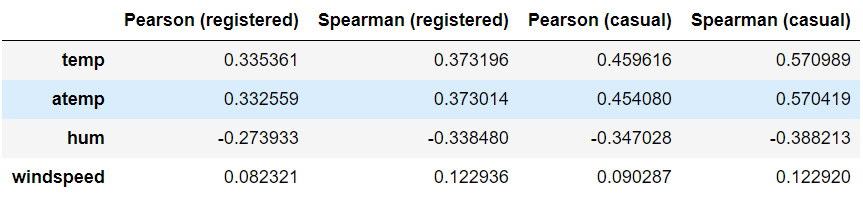Figure 1.26: The Pearson and Spearman correlation coefficients

Note

To access the source code for this specific section, please refer to https://packt.live/30OlyGW.

You can also run this example online at https://packt.live/3e7SmP2. You must execute the entire Notebook in order to get the desired result.

As we can observe, for most of the variables, the Pearson and Spearman correlation coefficient are close enough (some non-linearity is to be expected). The most striking difference between the two coefficients occurs when comparing the `temp` (and `atemp`) and `casual` columns. More precisely, the Spearman correlation is quite high, meaning that there is significant evidence for a nonlinear, relatively strong and positive relationship.

An interpretation of this result is that casual customers are far keener on using the bike sharing service when temperatures are higher. We have already seen from our previous analysis that casual customers ride mostly during the weekend, and they do not rely on bike sharing services for commuting to work. This conclusion is again confirmed by the strong relationship with temperature, as opposed to registered customers, whose rides have a weaker correlation with temperature.

### Correlation Matrix Plot

A useful technique when performing a comparison between different continuous features is the correlation matrix plot. It allows the analyst to quickly visualize any possible relationships between the different features and identify potential clusters with highly correlated features.

The next code snippet does that:

```# plot correlation matrix
cols = ["temp", "atemp", "hum", "windspeed", \
"registered", "casual"]
plot_data = preprocessed_data[cols]
corr = plot_data.corr()
fig = plt.figure(figsize=(10,8))
plt.matshow(corr, fignum=fig.number)
plt.xticks(range(len(plot_data.columns)), plot_data.columns)
plt.yticks(range(len(plot_data.columns)), plot_data.columns)
plt.colorbar()
plt.ylim([5.5, -0.5])
fig.savefig('figs/correlations.png', format='png')```

The output should be as follows: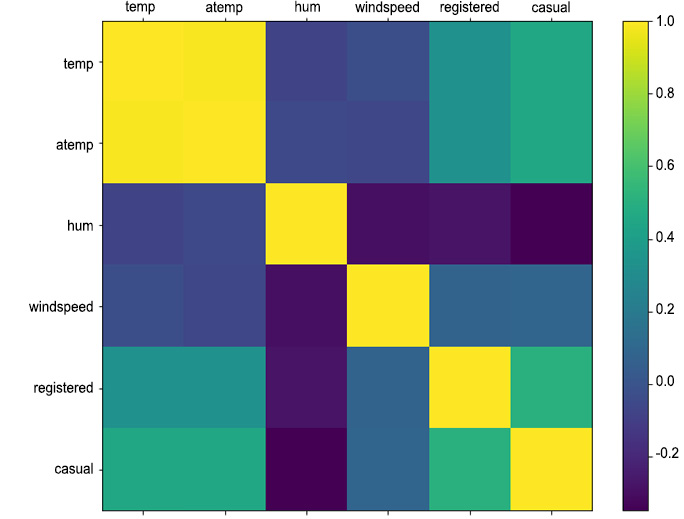Figure 1.27: Correlation matrix between continuous weather features and rides

This concludes our analysis of the weather columns and their impact on the number of rides. In the next section, we will exploit more advanced techniques for time-dependent features, known as time series analysis.

# Time Series Analysis

In this section, we perform a time series analysis on the rides columns (`registered` and `casual`) in the bike sharing dataset.

A time series is a sequence of observations equally spaced in time and in chronological order. Typical examples of time series are stock prices, yearly rainfall, or the number of customers using a specific transportation service every day. When observing time series their fluctuation might result in random values, but, often, they exhibit certain patterns (for example, the highs and lows of ocean tides or hotel prices in the proximity of fares).

When studying time series, an important concept is the notion of stationarity. A time series is said to be strongly stationary if all aspects of its behavior do not change in time. In other words, given a time series, `{Y`t`}`(t≥0) , for each `m` and `n`, the distributions of `Y`1`,…,Y`n and `Y`(m+1)`,…,Y`(m+n) are the same. In practice, strong stationarity is quite a restrictive assumption and, in most of the cases, not satisfied. Furthermore, for most of the methods illustrated later in this section to work, it is enough to have a time series that is weakly stationary, that is, its mean, standard deviation, and covariance are stationary with respect to time. More precisely, given `{Y`t`}`(t ≥ 0), we can observe:

• `E(Y`i` )=μ` (a constant) for every `i`
• `Var(Y`i` )=σ^2` (a constant) for every `i`
• `Corr(Y`i`,Y`j` )=ρ(i-j)` for every `i` and `j` and some function ρ(h)

In order to check stationarity in practice, we can rely on two different techniques for identifying time series stationarity: rolling statistics and augmented Dickey-Fuller stationarity test (in most cases, we consider both of them).

Rolling statistics is a practical method in which we plot the rolling mean and standard deviation of a time series and visually identify whether those values fluctuate around a constant one, without large deviations. We have to inform the reader that this is more a rule-of-thumb approach and not a rigorous statistical test for stationarity.

Augmented Dickey-Fuller stationarity test is a statistical test in which the null hypothesis is that the time series is nonstationary. Hence, when performing the test, a small p-value would be strong evidence against the time series being nonstationary.

Note

Since providing a detailed introduction to the augmented Dickey-Fuller test is out of the scope of this book, we refer the interested reader to the original book, Fuller, W. A. (1976). Introduction to Statistical Time Series. New York: John Wiley and Sons. ISBN 0-471-28715-6.

In practice, we rely on both of the techniques, as plotting the rolling statistics is not a rigorous approach. Let's define a utility function, which will perform both tests for us:

```"""
define function for plotting rolling statistics and ADF test for time series
"""
def test_stationarity(ts, window=10, **kwargs):
# create dataframe for plotting
plot_data = pd.DataFrame(ts)
plot_data['rolling_mean'] = ts.rolling(window).mean()
plot_data['rolling_std'] = ts.rolling(window).std()
# compute p-value of Dickey-Fuller test
ax = plot_data.plot(**kwargs)
ax.set_title(f"Dickey-Fuller p-value: {p_val:.3f}")```

We also need to extract the daily `registered` and `casual` rides from our preprocessed data:

```# get daily rides
daily_rides = preprocessed_data[["dteday", "registered", \
"casual"]]
daily_rides = daily_rides.groupby("dteday").sum()
# convert index to DateTime object
daily_rides.index = pd.to_datetime(daily_rides.index)```

Applying the previously defined `test_stationarity()` function to the daily rides produces the following plots: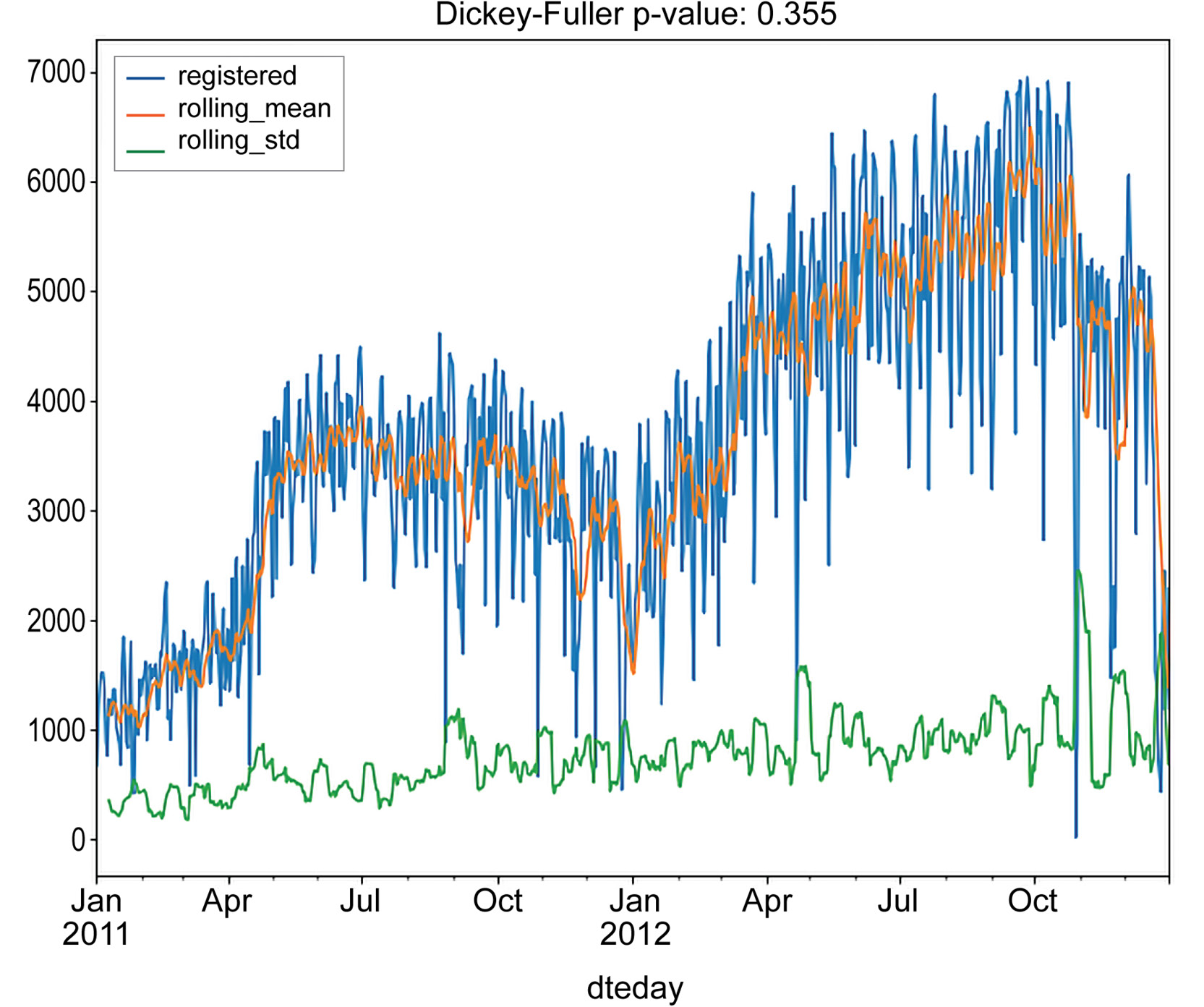Figure 1.28: Stationarity test results for aggregated daily registered rides

The output for daily casual rides can be as follows: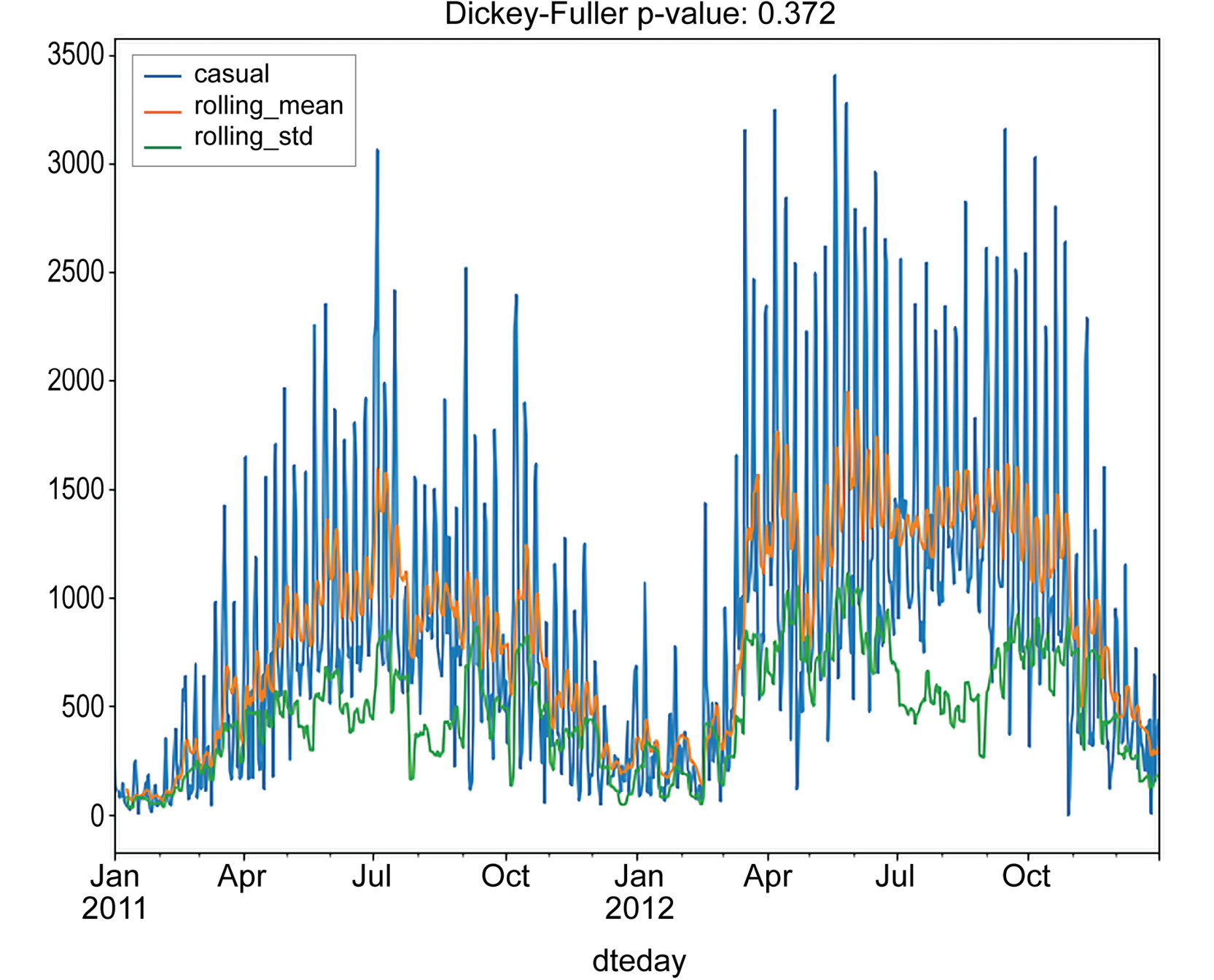Figure 1.29: Stationarity test results for aggregated daily casual rides

From the performed tests, we can see that neither the moving average nor standard deviations are stationary. Furthermore, the Dickey-Fuller test returns values of 0.355 and 0.372 for the `registered` and `casual` columns, respectively. This is strong evidence that the time series is not stationary, and we need to process them in order to obtain a stationary one.

A common way to detrend a time series and make it stationary is to subtract either its rolling mean or its last value, or to decompose it into a component that will contain its trend, seasonality, and residual components. Let's first check whether the time series is stationary by subtracting their rolling means and last values:

```# subtract rolling mean
registered = daily_rides["registered"]
registered_ma = registered.rolling(10).mean()
registered_ma_diff = registered - registered_ma
registered_ma_diff.dropna(inplace=True)
casual = daily_rides["casual"]
casual_ma = casual.rolling(10).mean()
casual_ma_diff = casual - casual_ma
casual_ma_diff.dropna(inplace=True)```

The resulting time series are tested for stationarity, and the results are shown in the following figures: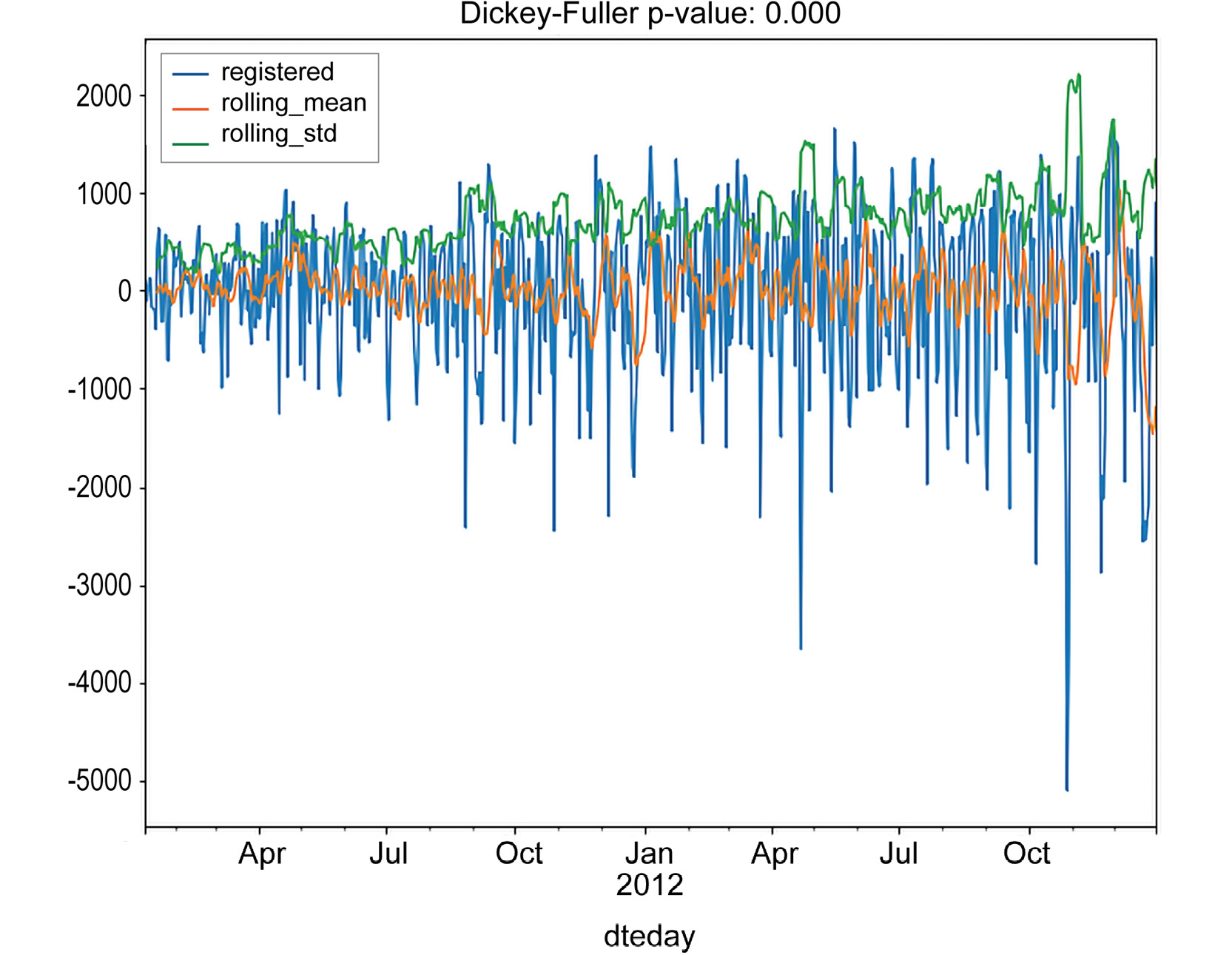Figure 1.30: Time series tested for stationarity for registered rides

The output for casual rides will be as follows: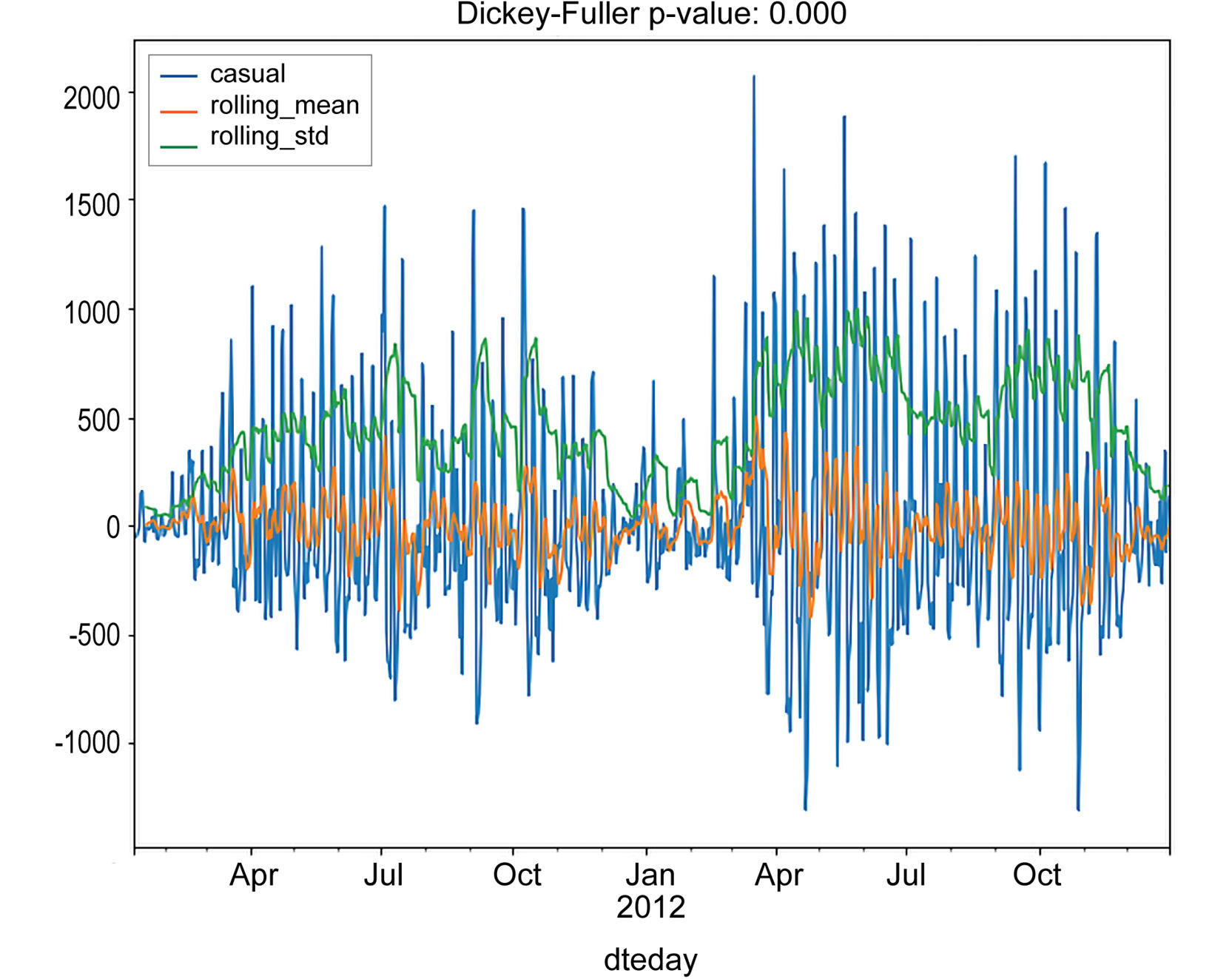Figure 1.31: Time series tested for stationarity for casual rides

Subtracting the last value can be done in the same way:

```# subtract last value
registered = daily_rides["registered"]
registered_diff = registered - registered.shift()
registered_diff.dropna(inplace=True)
casual = daily_rides["casual"]
casual_diff = casual - casual.shift()
casual_diff.dropna(inplace=True)
plt.figure()
test_stationarity(registered_diff, figsize=(10, 8))
plt.savefig('figs/daily_registered_diff.png', format='png')
plt.figure()
test_stationarity(casual_diff, figsize=(10, 8))
plt.savefig('figs/daily_casual_diff.png', format='png')```

The output should be as follows: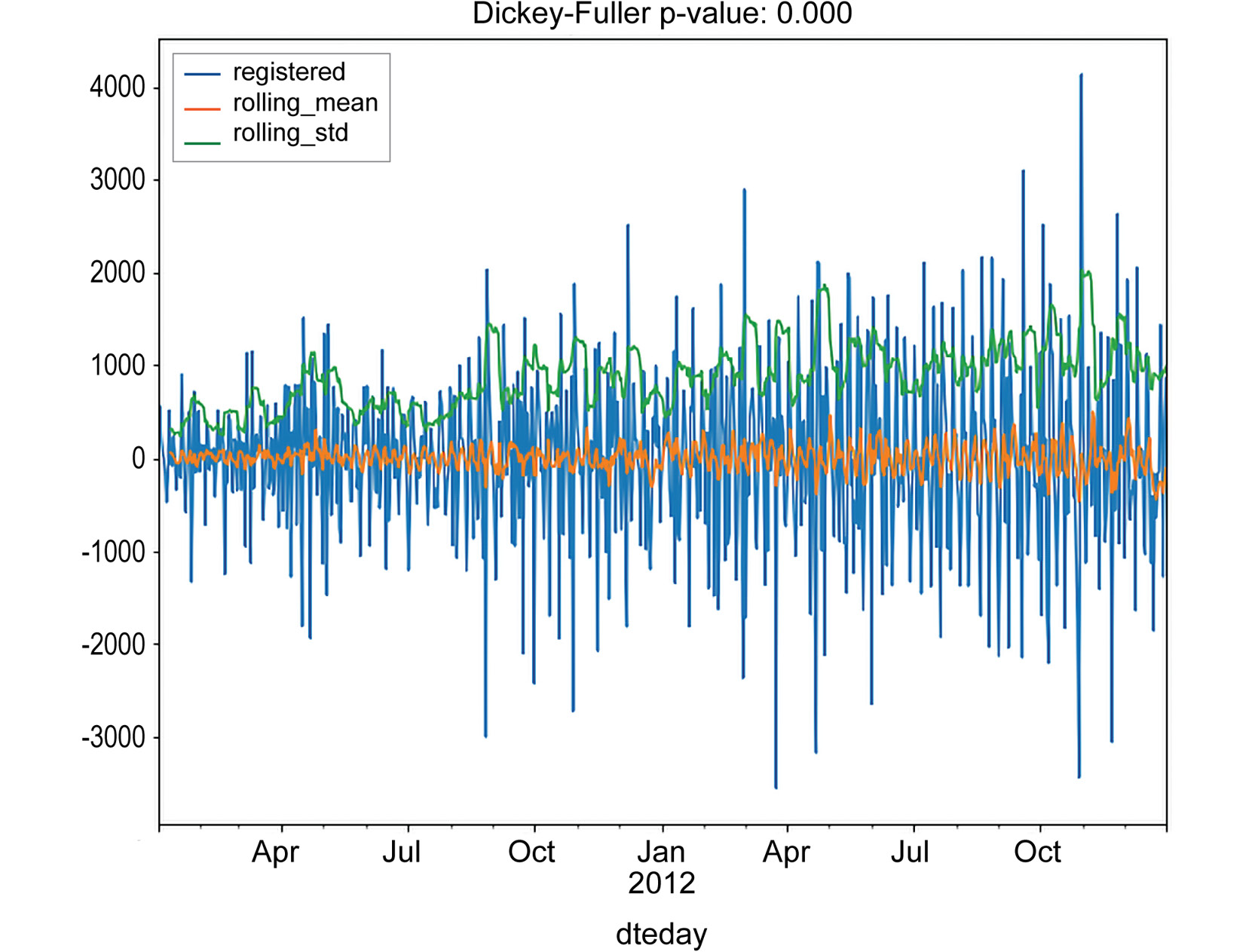Figure 1.32: Subtracting the last values from the time series for registered rides

The output for casual rides will be as follows: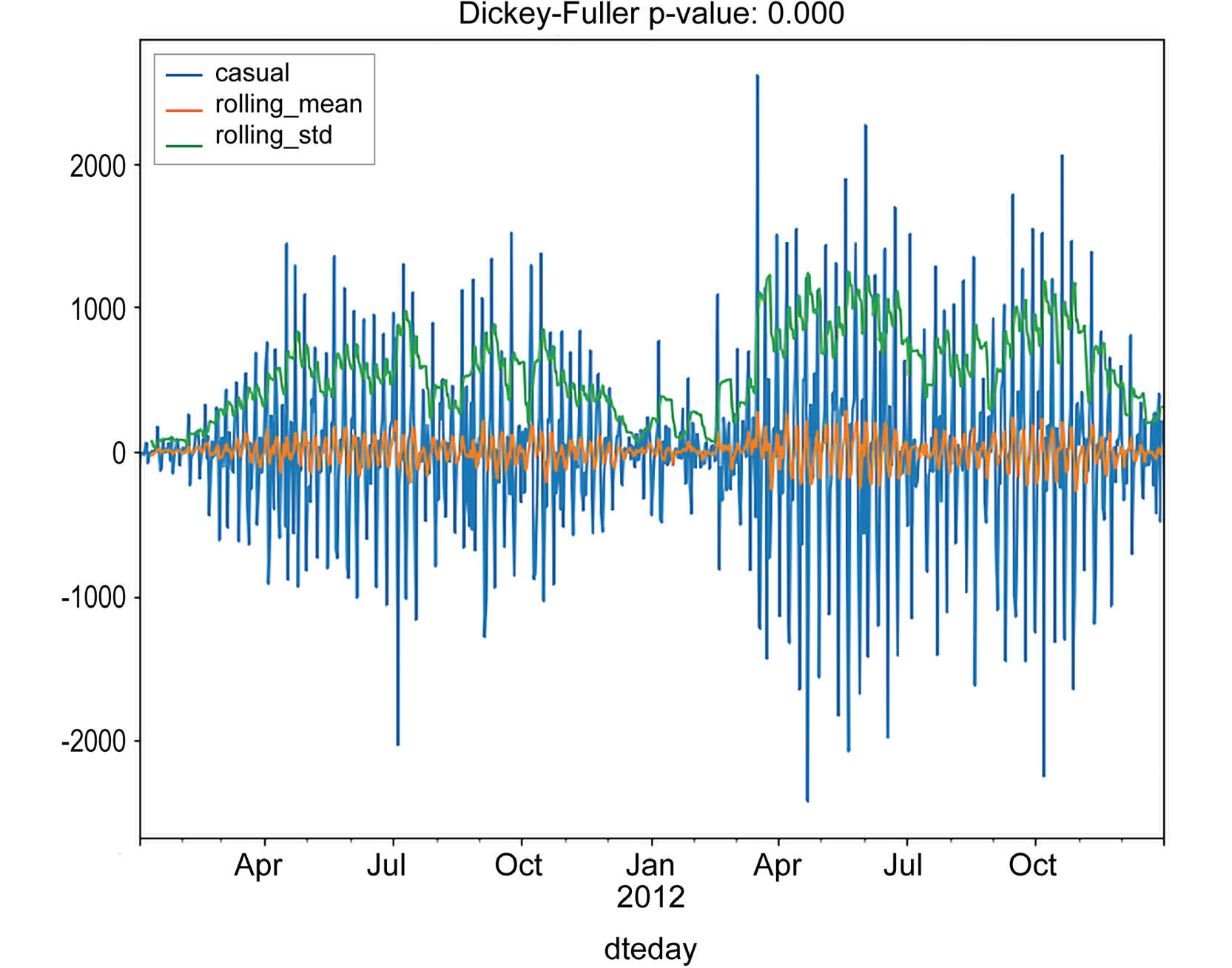Figure 1.33: Subtracting the last values from the time series for casual rides

As you can see, both of the techniques returned a time series, which is stationary, according to the Dickey-Fuller test. Note that an interesting pattern occurs in the casual series: a rolling standard deviation exhibits a clustering effect, that is, periods in which the standard deviation is higher and periods in which it is lower. This effect is quite common in certain fields (finance, for instance) and is known as volatility clustering. A possible interpretation, relative to our data, is that the number of casual rides increases during summer periods and drops during the winter.

As we saw from the last analysis, removing both the rolling mean and the last value returned a stationary time series. Let's also check also the previously mentioned technique, that is, time series decomposition. This involves breaking the original time series into separate components:

• Trend component: This component represents a long-term progression of the series. A trend component is present when there is a persistent increase or decrease in the series.
• Seasonal component: This component represents seasonality patterns in the data. A seasonal component persists when the data is influenced by certain seasonal factors (for example, monthly, quarterly, or yearly factors).
• Residual component: This component represents an irregular or noisy component. This component describes random fluctuations in the data, which are not captured by the other components. In general, this is the residual of the time series, that is, once the other components have been removed.

A time series decomposition can be framed in the following way. Let's denote, with `Y`t, the value of the original series at time instance `t`, and let `T`t`, S`t, and `R`t represent the trend, seasonal, and residual components, respectively. We will refer to the decomposition as additive if the following holds: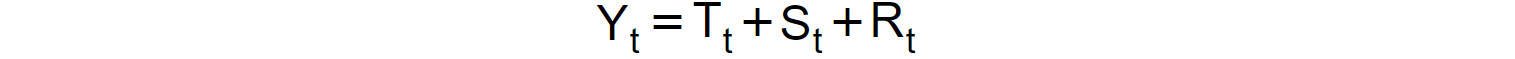And we will refer to the decomposition as multiplicative if the following holds: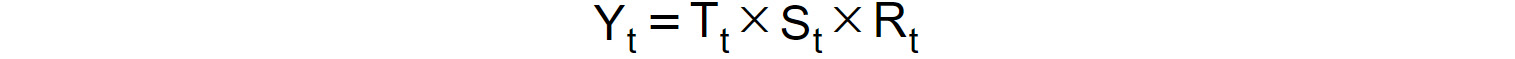Figure 1.35: Decomposition as multiplicative

In the following exercise, we will illustrate how to perform time series decomposition in Python.

## Exercise 1.06: Time Series Decomposition in Trend, Seasonality, and Residual Components

In this exercise, you will exploit seasonal decomposition in the `statsmodel` Python library in order to decompose the number of rides into three separate components, trend, seasonal, and residual components:

1. Use the `statsmodel.tsa.seasonal.` `seasonal_decompose()` method to decompose the `registered` and `casual` rides:
```from statsmodels.tsa.seasonal import seasonal_decompose
registered_decomposition = seasonal_decompose(\
daily_rides["registered"])
casual_decomposition = seasonal_decompose(daily_rides["casual"])```
2. To access each of these three signals, use `.trend`, `.seasonal`, and `.resid` variables. Furthermore, obtain visual results from the generated decompositions by calling the `.plot()` method:
```# plot decompositions
registered_plot = registered_decomposition.plot()
registered_plot.set_size_inches(10, 8)
casual_plot = casual_decomposition.plot()
casual_plot.set_size_inches(10, 8)
registered_plot.savefig('figs/registered_decomposition.png', \
format='png')
casual_plot.savefig('figs/casual_decomposition.png', \
format='png')```

The output for registered rides is as follows: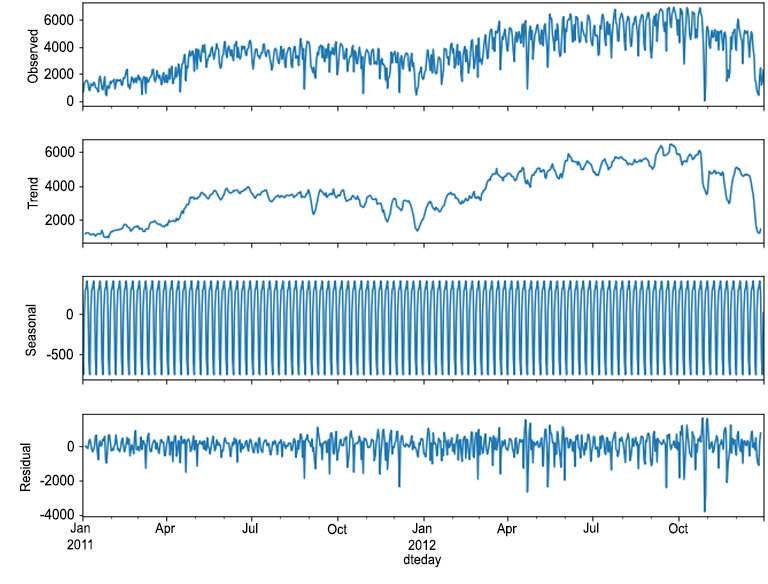Figure 1.36: Decomposition for registered rides

The output for casual rides will be as follows: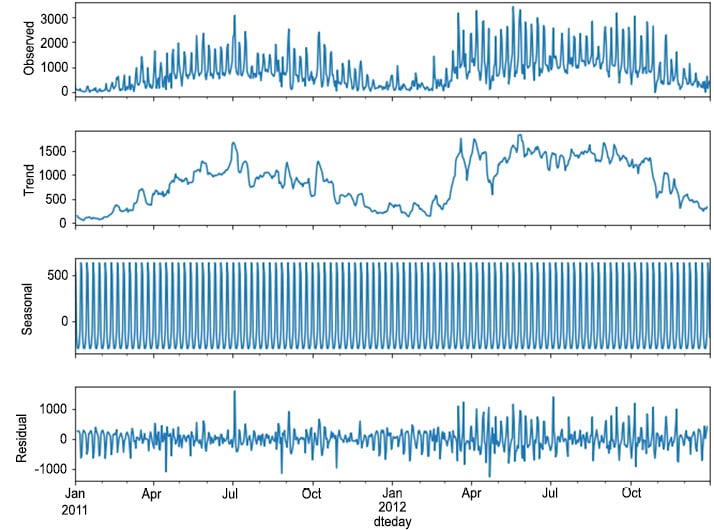Figure 1.37: Decomposition for casual rides

3. Test the residuals obtained for stationarity:
```# test residuals for stationarity
plt.figure()
test_stationarity(registered_decomposition.resid.dropna(), \
figsize=(10, 8))
plt.savefig('figs/registered_resid.png', format='png')
plt.figure()
test_stationarity(casual_decomposition.resid.dropna(), \
figsize=(10, 8))
plt.savefig('figs/casual_resid.png', format='png')```

The output will be as follows: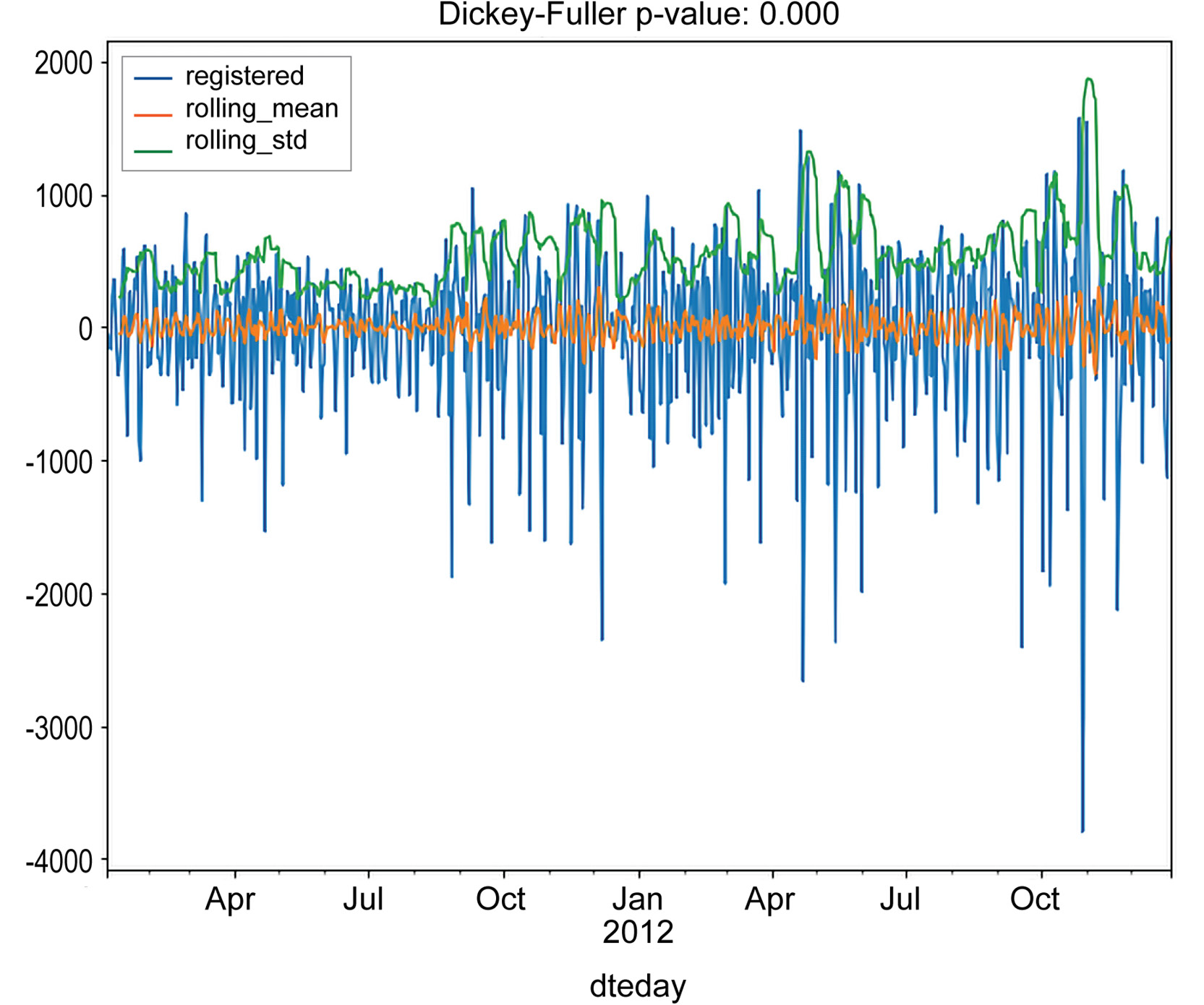Figure 1.38: Test residuals for stationarity for registered rides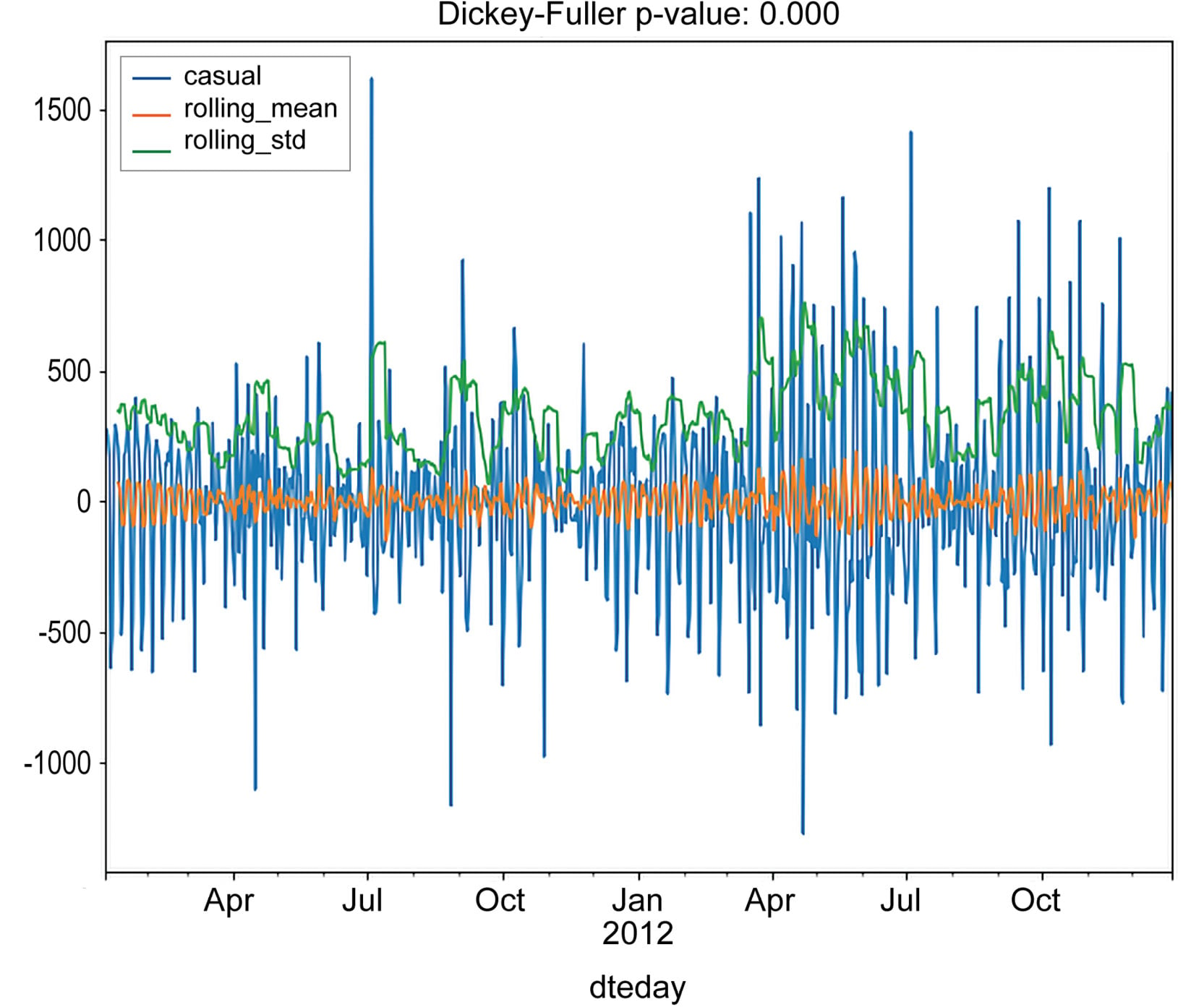Figure 1.39: Test residuals for stationarity for casual rides

As you can see, the residuals satisfy our stationary test.

Note

To access the source code for this specific section, please refer to https://packt.live/3hB8871.

You can also run this example online at https://packt.live/2BaXsLJ. You must execute the entire Notebook in order to get the desired result.

A common approach to modeling a time series is to assume that past observations somehow influence future ones. For instance, customers who are satisfied by using the bike sharing service will more likely recommend it, producing, in this way, a positive impact on the service and a higher number of customers (obviously, any negative feedback has the opposite effect, reducing the number of customers). Hence, increasing the number of customers and the quality of the service increases the number of recommendations and, therefore, the number of new customers. In this way, a positive feedback loop is created, in which the current number of rides correlates with its past values. These types of phenomena are the topic of the next section.

# ARIMA Models

1. Autoregressive Integrated Moving Average (ARIMA) models are a class of statistical models that try to explain the behavior of a time series using its own past values. Being a class of models, ARIMA models are defined by a set of parameters (p,d,q), each one corresponding to a different component of the ARIMA model:

• Autoregressive of order p: An autoregressive model of order p (AR(p) for short) models the current time series entry as a linear combination of its last p values. The mathematical formulation is as follows:Figure 1.40: Expression for an autoregressive model of order p

Here, `α` is the intercept term, `Y`(t-i) is the lag-I term of the series with the respective coefficient `β`i, while `ϵ`t is the error term (that is, the normally distributed random variable with mean 0 and variance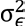).

• Moving average of order q: A moving average model of order q (MA(q) for short) attempts to model the current value of the time series as a linear combination of its past error terms. Mathematically speaking, it has the following formula:Figure 1.41: Expression for the moving average model of order q

As in the autoregressive model, `α` represents a bias term; `ϕ`1,…,`ϕ`q are parameters to be estimated in the model; and `ϵ`t,…,`ϵ`(t-q) are the error terms at times `t,…,t-q`, respectively.

• Integrated component of order d: The integrated component represents a transformation in the original time series, in which the transformed series is obtained by getting the difference between `Y`t and `Y`(t-d), hence the following: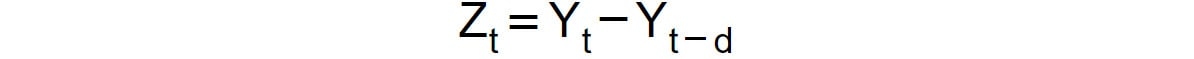Figure 1.42: Expression for an integrated component of order d

The integration term is used for detrending the original time series and making it stationary. Note that we already saw this type of transformation when we subtracted the previous entry in the number of rides, that is, when we applied an integration term of order 1.

In general, when we apply an ARIMA model of order (p,d,q) to a time series, `{Y`t`}` , we obtain the following model:

1. First, integrate the original time series of order d, and then obtain the new series:Figure 1.43: Integrating the original time series

2. Then, apply a combination of the AR(p) and MA(q) models, also known as the autoregressive moving average model, or ARMA(p,q), to the transformed series, `{Z`t `}`t:Figure 1.44: Expression for ARMA

Here, the coefficients `α,β`1`,…,β`p`,ϕ`1`,…,ϕ`q` `are to be estimated.

A standard method for finding the parameters (p,d,q) of an ARIMA model is to compute the autocorrelation and partial autocorrelation functions (ACF and PACF for short). The autocorrelation function measures the Pearson correlation between the lagged values in a time series as a function of the lag:Figure 1.45: Autocorrelation function as a function of the lag

In practice, the ACF measures the complete correlation between the current entry, `Y`t, and its past entries, lagged by `k` . Note that when computing the ACF(k), the correlation between `Y`t with all intermediate values (`Y`(t-1)`,…,Y`(t-k+1)) is not removed. In order to account only for the correlation between and `Y`(t-k), we often refer to the PACF, which only measures the impact of `Y`(t-k) on `Y`t.

ACF and PACF are, in general, used to determine the order of integration when modeling a time series with an ARIMA model. For each lag, the correlation coefficient and level of significance are computed. In general, we aim at an integrated series, in which only the first few lags have correlation greater than the level of significance. We will demonstrate this in the following exercise.

## Exercise 1.07: ACF and PACF Plots for Registered Rides

In this exercise, we will plot the autocorrelation and partial autocorrelation functions for the `registered` number of rides:

1. Access the necessary methods for plotting the ACF and PACF contained in the Python package, `statsmodels`:
`from statsmodels.graphics.tsaplots import plot_acf, plot_pacf`
2. Define a 3 x 3 grid and plot the ACF and PACF for the original series of registered rides, as well as for its first- and second-order integrated series:
```fig, axes = plt.subplots(3, 3, figsize=(25, 12))
# plot original series
original = daily_rides["registered"]
axes[0,0].plot(original)
axes[0,0].set_title("Original series")
plot_acf(original, ax=axes[0,1])
plot_pacf(original, ax=axes[0,2])
# plot first order integrated series
first_order_int = original.diff().dropna()
axes[1,0].plot(first_order_int)
axes[1,0].set_title("First order integrated")
plot_acf(first_order_int, ax=axes[1,1])
plot_pacf(first_order_int, ax=axes[1,2])
# plot first order integrated series
second_order_int = first_order_int.diff().dropna()
axes[2,0].plot(first_order_int)
axes[2,0].set_title("Second order integrated")
plot_acf(second_order_int, ax=axes[2,1])
plot_pacf(second_order_int, ax=axes[2,2])```

The output should be as follows: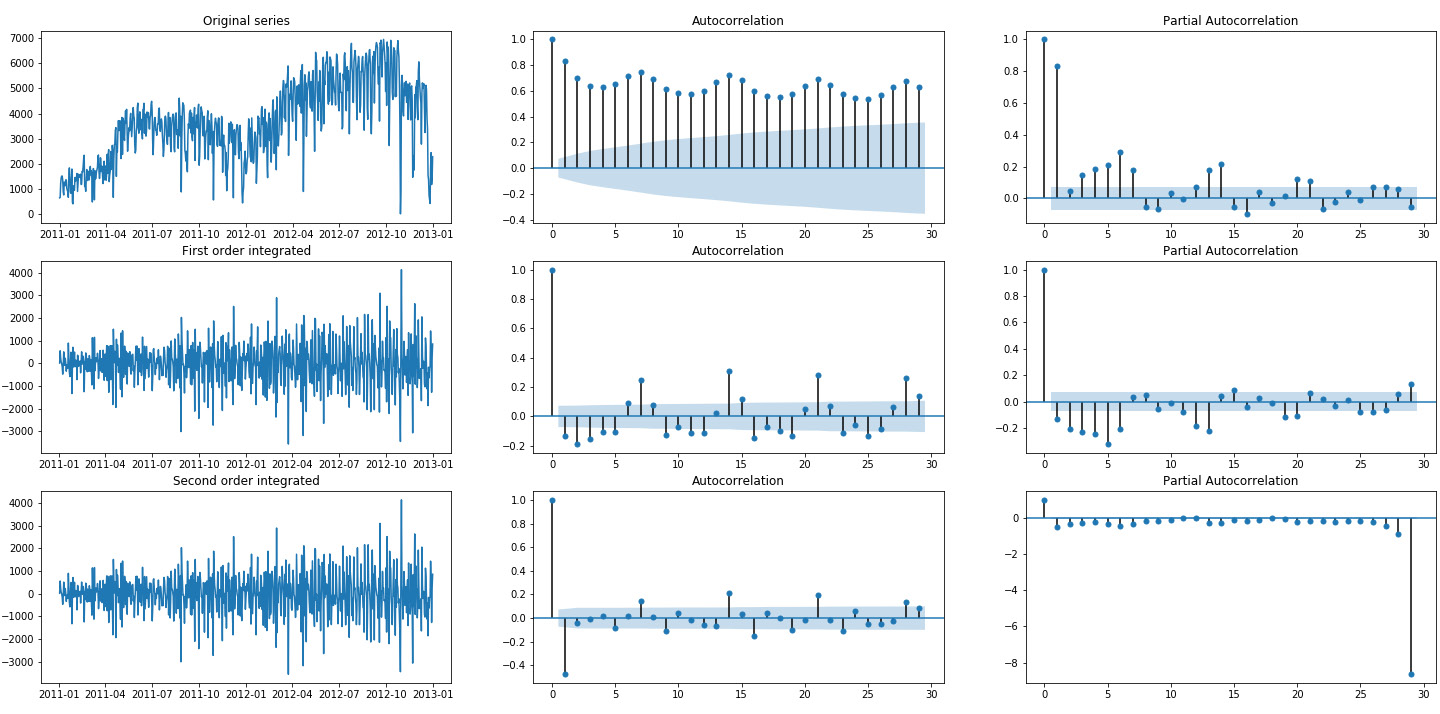Figure 1.46: Autocorrelation and partial autocorrelation plots of registered rides

As you can see from the preceding figure, the original series exhibits several autocorrelation coefficients that are above the threshold. The first order integrated series has only a few, which makes it a good candidate for further modeling (hence, selecting an ARIMA(p,1,q) model). Finally, the second order integrated series present a large negative autocorrelation of lag 1, which, in general, is a sign of too large an order of integration.

Now focus on finding the model parameters and the coefficients for an ARIMA(p,d,q) model, based on the observed registered rides. The general approach is to try different combinations of parameters and chose the one that minimizes certain information criterion, for instance, the Akaike Information Criterion (AIC) or the Bayesian Information Criterion (BIC):

Akaike Information Criterion:Figure 1.47: Expression for AIC

Bayesian Information Criterion:Figure 1.48: Expression for BIC

Here, `k` is the number of parameters in the selected model, `n` is the number of samples, and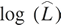is the log likelihood. As you can see, there is no substantial difference between the two criteria and, in general, both are used. If different optimal models are selected according to the different IC, we tend to find a model in between.

3. In the following code snippet, fit an ARIMA(p,d,q) model to the registered column. Note that the `pmdarima` package is not a standard in Anaconda; therefore, in order to install it, you need to install it via the following:
`conda install -c saravji pmdarima`

And then perform the following:

```from pmdarima import auto_arima
model = auto_arima(registered, start_p=1, start_q=1, \
max_p=3, max_q=3, information_criterion="aic")
print(model.summary())```

Python's `pmdarima` package has a special function that automatically finds the best parameters for an ARIMA(p,d,q) model based on the AIC. Here is the resulting model: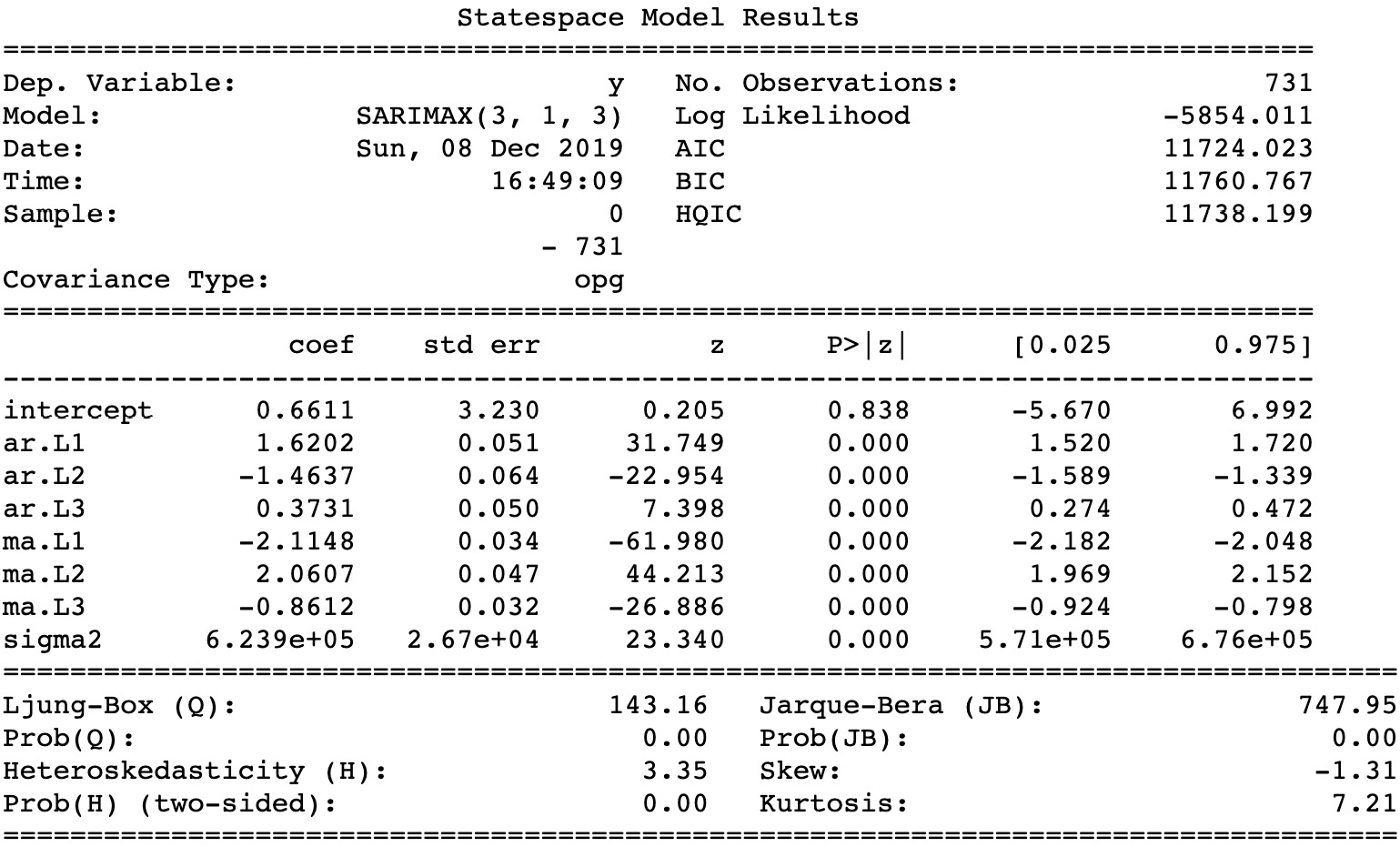Figure 1.49: The resulting model based on AIC

As you can see, the best selected model was ARIMA(3,1,3), with the `coef` column containing the coefficients for the model itself.

4. Finally, evaluate how well the number of rides is approximated by the model by using the `model.predict_in_sample()` function:
```# plot original and predicted values
plot_data = pd.DataFrame(registered)
plot_data['predicted'] = model.predict_in_sample()
plot_data.plot(figsize=(12, 8))
plt.ylabel("number of registered rides")
plt.title("Predicted vs actual number of rides")
plt.savefig('figs/registered_arima_fit.png', format='png')```

The output should be as follows: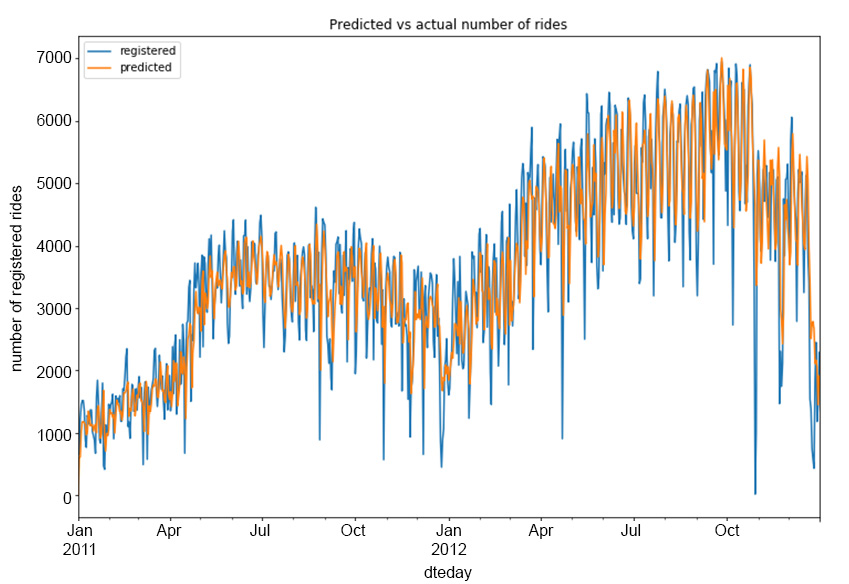Figure 1.50: Predicted versus the actual number of registered rides

As you can see, the `predicted` column follows the original one quite well, although it is unable to correctly model a large number of rise and fall movements in the `registered` series.

Note

To access the source code for this specific section, please refer to https://packt.live/37xHHKQ.

You can also run this example online at https://packt.live/30MqcFf. You must execute the entire Notebook in order to get the desired result.

In this first chapter, we presented various techniques from data analysis and statistics, which will serve as a basis for further analysis in the next chapter, in order to improve our results and to obtain a better understanding of the problems we are about to deal with.

## Activity 1.01: Investigating the Impact of Weather Conditions on Rides

In this activity, you will investigate the impact of weather conditions and their relationship with the other weather-related columns (`temp`, `atemp`, `hum`, and `windspeed`), as well as their impact on the number of registered and casual rides. The following steps will help you to perform the analysis:

1. Import the initial `hour` data.
2. Create a new column in which `weathersit` is mapped to the four categorical values specified in Exercise 1.01, Preprocessing Temporal and Weather Features. (`clear`, `cloudy`, `light_rain_snow`, and `heavy_rain_snow`).
3. Define a Python function that accepts as input the hour data, a column name, and a weather condition, and then returns a seaborn `regplot` in which regression plots are produced between the provided column name and the registered and casual rides for the specified weather condition.
4. Produce a 4 x 4 plot in which each column represents a specific weather condition (`clear`, `cloudy`, `light_rain_snow`, and `heavy_rain_snow`), and each row of the specified four columns (`temp`, `atemp`, `hum`, and `windspeed`). A useful function for producing the plot might be the `matplotlib.pyplot.subplot()` function.

Note

For more information on the `matplotlib.pyplot.subplot()` function, refer to https://pythonspot.com/matplotlib-subplot/.

5. Define a second function that accepts the hour data, a column name, and a specific weather condition as an input, and then prints the Pearson's correlation and p-value between the registered and casual rides and the provided column for the specified weather condition (once when the correlation is computed between the registered rides and the specified column and once between the casual rides and the specified column).
6. Iterating over the four columns (`temp`, `atemp`, `hum`, and `windspeed`) and four weather conditions (`clear`, `cloudy`, `light_rain_snow`, and `heavy_rain_snow`), print the correlation for each column and each weather condition by using the function defined in Step 5.

The output should be as follows: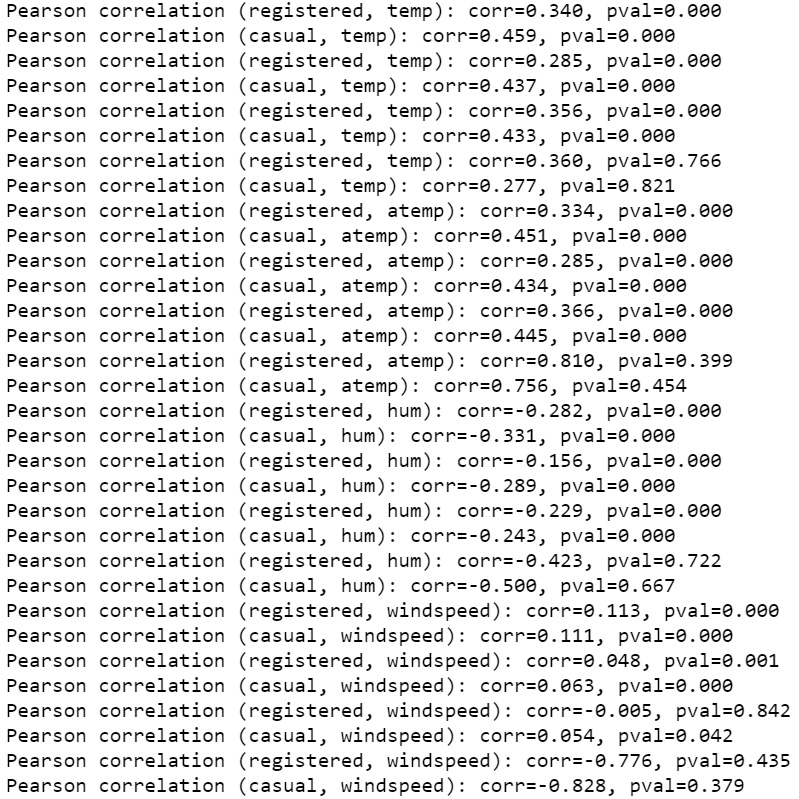Figure 1.51: Correlation between weather and the registered/casual rides

Note

The solution for this activity can be found via this link.

# Summary

In this chapter, we studied a business problem related to bike sharing services. We started by presenting some of the main visual techniques in data analysis, such as bar plots, scatter plots, and time series visualizations. We also analyzed customer behaviors based on different time frames and weather conditions. We introduced the reader to hypothesis testing and some of its main applications. Finally, we presented the basics of time series analysis, and how to identify the best time series models when dealing with nonstationary time series.

In the next chapter, we will proceed with our analysis by presenting some of the commonly used data transformation techniques, topics from probability theory, and further techniques in hypothesis testing.

• ##### Gururajan Govindan

Gururajan Govindan is a data scientist, intrapreneur, and trainer with more than seven years of experience working across domains such as finance and insurance. He is also an author of The Data Analysis Workshop, a book focusing on data analytics. He is well known for his expertise in data-driven decision-making and machine learning with Python.

Browse publications by this author
• ##### Shubhangi Hora

Shubhangi Hora is a data scientist, Python developer, and published writer. With a background in computer science and psychology, she is particularly passionate about healthcare-related AI, including mental health. Shubhangi is also a trained musician.

Browse publications by this author
• ##### Konstantin Palagachev

Konstantin Palagachev holds a Ph.D. in applied mathematics and optimization, with an interest in operations research and data analysis. He is recognized for his passion for delivering data-driven solutions and expertise in the area of urban mobility, autonomous driving, insurance, and finance. He is also a devoted coach and

Browse publications by this author

### Latest Reviews

(2 reviews total)